## 《算法和数据结构》算法篇_算法是数据的增删改查 英雄哪里出来_英雄哪里出来的博客-程序员宅基地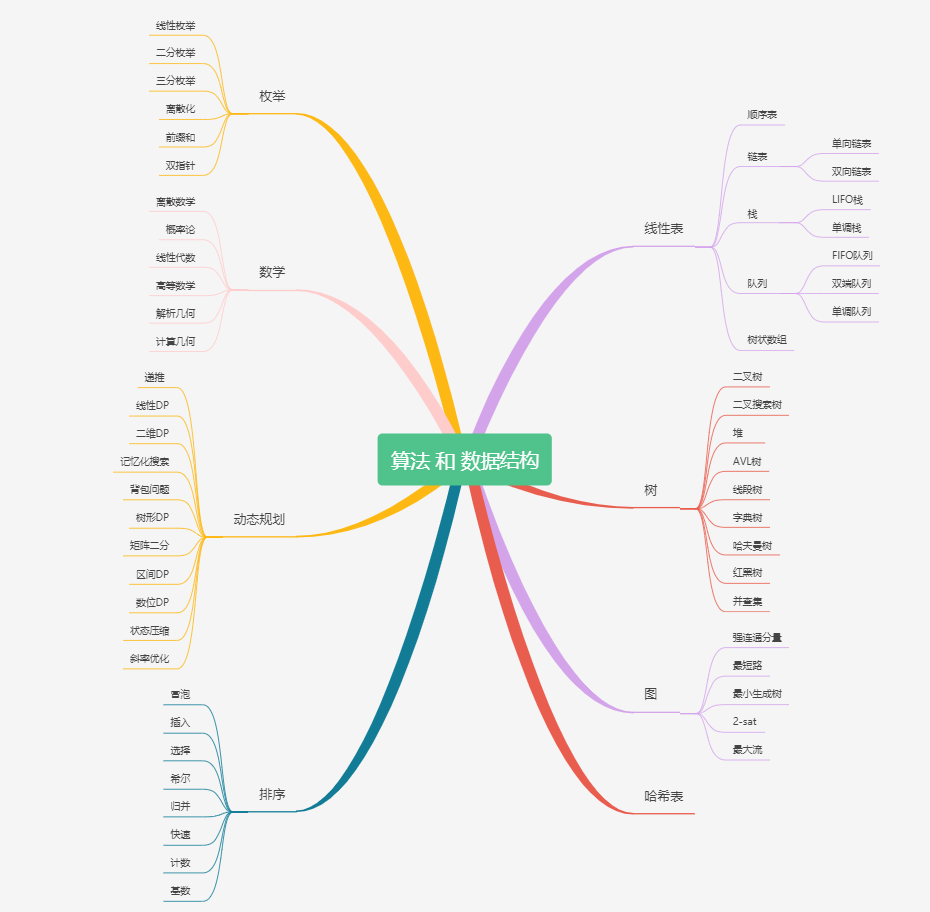# 前言

我大学的时候比较疯狂，除了上课的时候，基本都是在机房刷题，当然，有时候连上课都在想题目，纸上写好代码，一下课就冲进机房把代码敲了，目的很单纯，为了冲排行榜，就像玩游戏一样，享受霸榜的快感。
当年主要是在 「 杭电OJ 」「 北大OJ 」 这两个在线平台上刷题，那时候还没有（「 LeetCode 」「 牛客 」「 洛谷 」 这些先如今非常的刷题网站），后来参加工作以后，剩余的时间不多了，也就没怎么刷了， 但是 「 算法思维 」 也就是靠上大学那「 四年 」锻炼出来的。当年题目少，刷题的人也少，所以勉强还冲到过第一，现在去看已经 58 名了，可见 「 长江后浪推前浪 」，前浪 S 在沙滩上。时势造英雄啊！北大人才辈出，相对题目也比较难，所以明显有点 「 心有余而力不足 」 的感觉，刷的相对就少很多，而且这个 OJ 也没什么人维护了，看我的签名，当时竟然还想着给博客引点流，现在估计都没什么人去那个网站了吧。

这篇文章的主要目的是为尚未接触算法的朋友提供一个学习算法的路线指引，但是实际学习过程还是需要看个人的毅力和坚持。那么，让我们先来看下需要按照什么样的顺序来进行刷题吧。下图代表的是 LeetCode 经典的算法和数据结构的总纲。相信看我文章的大多数都是「 大学生 」，能上大学的都是「 精英 」，那么我们自然要「 精益求精 」，如果你还是「 大一 」，那么太好了，你拥有大把时间，当然你可以选择「 刷剧 」，然而，「 学好算法 」，三年后的你自然「 不能同日而语 」
那么这里，我整理了「 几十个基础算法 」 的分类，点击开启：

如果链接被屏蔽，或者有权限问题，可以私聊作者解决。# 一、算法

## 1、排序### 1）冒泡排序■ 的柱形 代表尚未排好序的数
■ 的柱形 代表正在执行比较的两个数
■ 的柱形 代表已经排好序的数

我们看到，首先需要将 「第一个元素」「第二个元素」 进行 「比较」，如果 前者 大于 后者，则进行 「交换」，然后再比较 「第二个元素」「第三个元素」 ，以此类推，直到 「最大的那个元素」 被移动到 「最后的位置」
然后，进行第二轮「比较」，直到 「次大的那个元素」 被移动到 「倒数第二的位置」
最后，经过一定轮次的「比较」「交换」之后，一定可以保证所有元素都是 「升序」 排列的。

### 2）选择排序■ 的柱形 代表尚未排好序的数
■ 的柱形 代表正在执行 比较 的数
■ 的柱形 代表已经排好序的数
■ 的柱形 有两种：1、记录最小元素 2、执行交换的元素

我们发现，首先从 「第一个元素」「最后一个元素」 中选择出一个 「最小的元素」，和 「第一个元素」 进行 「交换」
然后，从 「第二个元素」「最后一个元素」 中选择出一个 「最小的元素」，和 「第二个元素」 进行 「交换」
最后，一定可以保证所有元素都是 「升序」 排列的。

### 3）插入排序■ 的柱形 代表尚未排好序的数
■ 的柱形 代表正在执行 比较 和 移动 的数
■ 的柱形 代表已经排好序的数
■ 的柱形 代表待执行插入的数

我们看到，首先需要将 「第二个元素」「第一个元素」 进行 「比较」，如果 前者 小于等于 后者，则将 后者 进行向后 「移动」前者 则执行插入；
然后，进行第二轮「比较」，即 「第三个元素」「第二个元素」、「第一个元素」 进行 「比较」， 直到 「前三个元素」 保持有序 。
最后，经过一定轮次的「比较」「移动」之后，一定可以保证所有元素都是 「升序」 排列的。

### 4）希尔排序一下子看完不是很理解，没有关系，我们把这几个过程分拆开来。第一趟分解后，如图所示：增量为 4，所有元素总共分为 4 组，分别为 [8, 3][5, 7][6, 10][4, 2]，同组内部分别执行插入排序，得到 [3, 8][5, 7][6, 10][2, 4]（由于每组只有两个元素，所以升序的情况位置不变，降序的情况执行组内元素位置交换，抖动一下代表保持原顺序不变，有一种 “我不换 ~~ 我不换” 的意思在里面 ）。

第二趟分解后，如图所示：增量为 2，所有元素总共分为 2 组，分别为 [3, 6, 8, 10][5, 2, 7, 4]，同组内部分别执行插入排序，[3, 6, 8, 10]已经升序，保持原样；[5, 2, 7, 4] 执行三次插入排序后变成 [2, 4, 5, 7]
第三趟分解后，如图所示：增量为 1，所有元素归为 1 组，为 [3, 2, 6, 4, 8, 5, 10, 7]。对它执行简单插入排序，执行完毕后，必然可以保证所有元素有序。

### 5）归并排序■ 的柱形 代表尚未排好序的数
■ 的柱形 代表已经排好序的数

我们发现，首先将 「 8个元素 」 分成 「 4个元素 」，再将 「 4个元素 」 分成 「 2个元素 」，然后 「比较」「 2个元素 」的值，使其在自己的原地数组内有序，然后两个 「 2个元素 」 的数组归并变成 「 4个元素 」 「升序」数组，再将两个「 4个元素 」 的数组归并变成 「 8个元素 」 「升序」数组。

### 6）快速排序■ 的柱形 代表尚未排好序的数
■ 的柱形 代表随机选定的基准数
■ 的柱形 代表已经排序好的数
■ 的柱形 代表正在遍历比较的数
■ 的柱形 代表比基准数小的数
■ 的柱形 代表比基准数大的数

我们发现，首先随机选择了一个 7 作为「 基准数 」，并且将它和最左边的数交换。然后往后依次遍历判断，小于 7 的数为 「 绿色 」 ，大于 7 的数为「 紫色 」，遍历完毕以后，将 7 和 「 下标最大的那个比 7 小的数 」交换位置，至此，7的左边位置上的数都小于它，右边位置上的数都大于它，左边和右边的数继续递归求解即可。

### 7）计数排序■ 的柱形 计数为 0 的数
■ 的柱形 计数为 1 的数
■ 的柱形 计数为 2 的数
■ 的柱形 计数为 3 的数
■ 的柱形 计数为 4 的数

我们看到，首先程序生成了一个区间范围为 [ 1 , 9 ] [1, 9] 「 计数器数组 」，并且一开始所有值的计数都为 0。
然后，遍历枚举「 原数组 」的所有元素，在 元素值 对应的计数器上执行 「 计数 」 操作。
最后，遍历枚举「 计数器数组 」，按照数组中元素个数放回到 「 原数组 」 中。这样，一定可以保证所有元素都是 「升序」 排列的。

### 8）基数排序「 红色的数字位 」 代表需要进行 「 哈希 」 映射到给定 「 队列 」 中的数字位。
我们看到，首先程序生成了一个区间范围为 [ 0 , 9 ] [0, 9] 「 基数队列 」
然后，总共进行了 4 轮「 迭代 」（因为最大的数总共 4 个数位）。
每次迭代，遍历枚举 「 原数组 」 中的所有数，并且取得本次迭代对应位的数字，通过「 哈希 」，映射到它「 对应的队列 」中 。然后将 「 队列 」 中的数据按顺序塞回 「 原数组 」 完成一次「 迭代 」，4 次「 迭代 」后，一定可以保证所有元素都是 「升序」 排列的。

## 2、枚举

### 1）线性枚举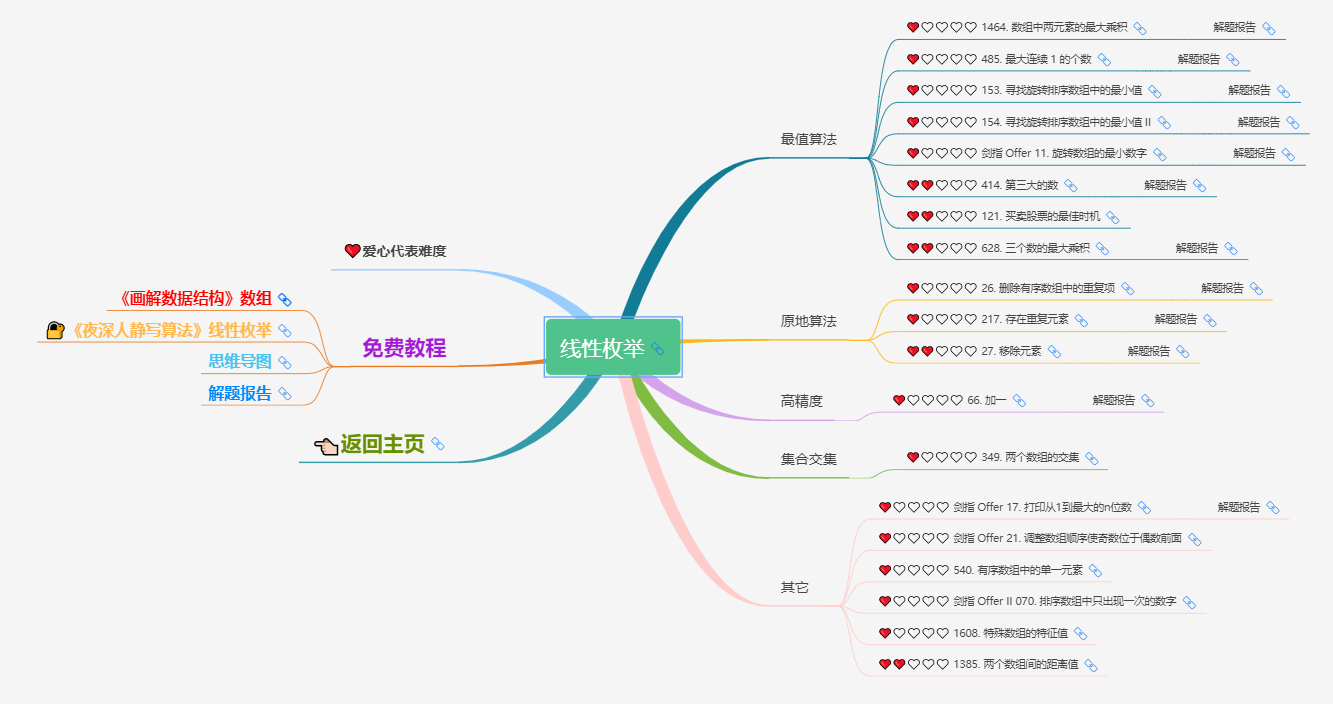线性枚举，一般配合的 数据结构 是 【数组】 或者 【链表】，实现方式就是一个循环。正因为只有一个循环，所以线性枚举解决的问题一般比较简单，而且很容易从题目中看出来。

编写一个函数，将输入的字符串反转过来。输入字符串以字符数组 char[] 的形式给出。

样例输入： [ “ a ” , “ b ” , “ c ” , “ d ” ] [“a”, “b”, “c”, “d”]
样例输出： [ “ d ” , “ c ” , “ b ” , “ a ” ] [ “d”, “c”, “b”, “a”]
原题出处： LeetCode 344. 反转字符串

翻转的含义，相当于就是 第一个字符 和 最后一个交换，第二个字符 和 最后第二个交换，… 以此类推，所以我们首先实现一个交换变量的函数 swap，然后再枚举 第一个字符、第二个字符、第三个字符 …… 即可。
对于第 i i 个字符，它的交换对象是 第 l e n − i − 1 len-i-1 个字符 (其中 l e n len 为字符串长度)。swap函数的实现，可以参考：《C语言入门100例》 - 例2 | 交换变量
线性枚举的过程为 O ( n ) O(n) ，交换变量为 O ( 1 ) O(1) ，两个过程是相乘的关系，所以整个算法的时间复杂度为 O ( n ) O(n)

class Solution {

public:
void swap(char& a, char& b) {
// (1)
char tmp = a;
a = b;
b = tmp;
}
void reverseString(vector<char>& s) {

int len = s.size();
for(int i = 0; i < len / 2; ++i) {
// (2)
swap(s[i], s[len-i-1]);
}
}
};

• ( 1 ) (1) 实现一个变量交换的函数，其中&是C++中的引用，在函数传参是经常用到，被称为：引用传递(pass-by-reference)，即被调函数的形式参数虽然也作为局部变量在堆栈中开辟了内存空间
，但是这时存放的是由主调函数放进来的实参变量的地址。被调函数对形参的任何操作都被处理成间接寻址，即通过堆栈中存放的地址访问主调函数中的实参变量。

• ( 2 ) (2) 这一步是做的线性枚举，注意枚举范围是 [ 0 , l e n / 2 − 1 ] [0, len/2-1]

### 2）二分枚举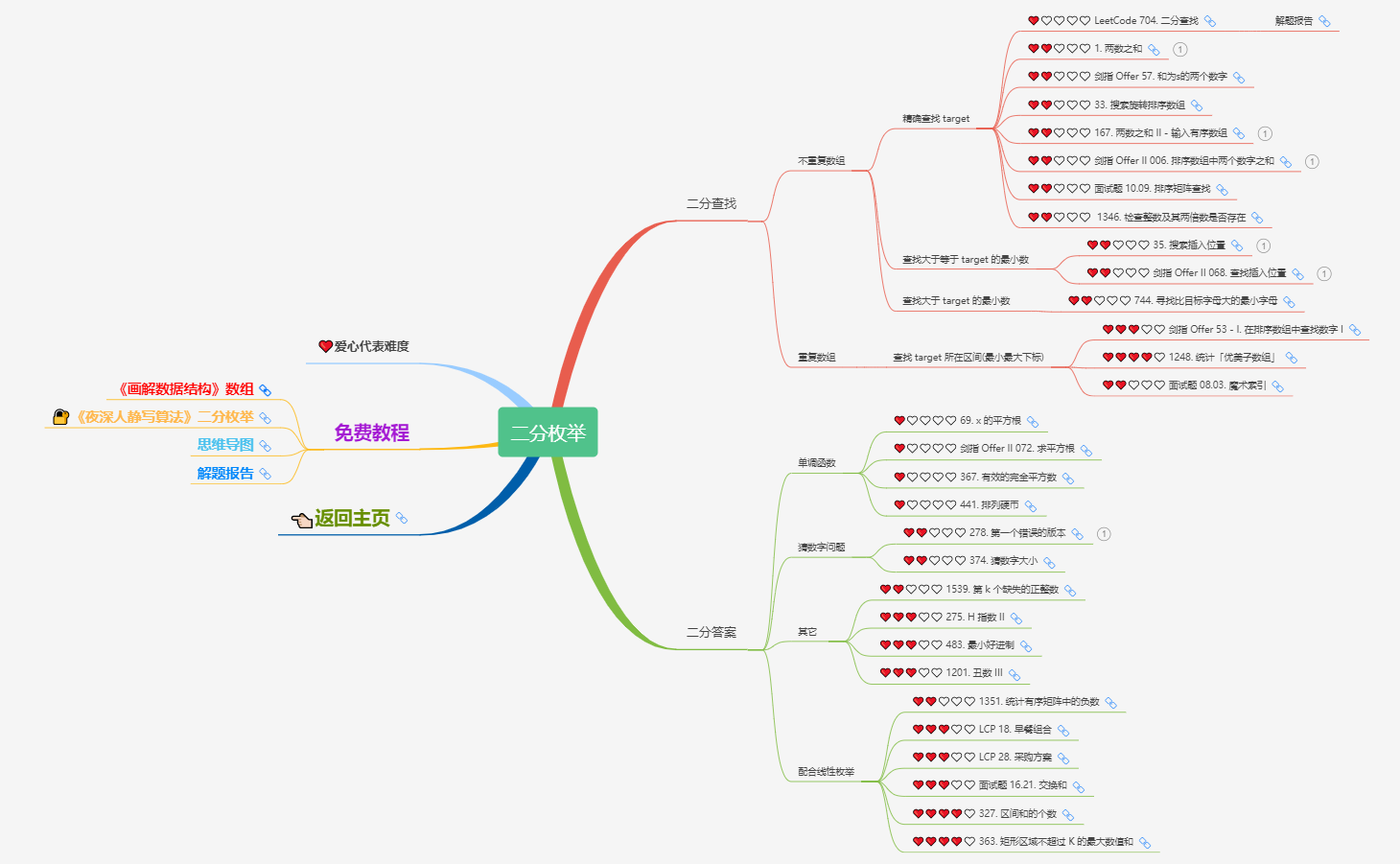能用二分枚举的问题，一定可以用线性枚举来实现，只是时间上的差别，二分枚举的时间复杂度一般为对数级，效率上会高不少。同时，实现难度也会略微有所上升。我们通过平时开发时遇到的常见问题来举个例子。

软件开发的时候，会有版本的概念。由于每个版本都是基于之前的版本开发的，所以错误的版本之后的所有版本都是错的。假设你有 n n 个版本 [ 1 , 2 , . . . , n ] [1, 2, ..., n] ，你想找出导致之后所有版本出错的第一个错误的版本。可以通过调用bool isBadVersion(version)接口来判断版本号version是否在单元测试中出错。实现一个函数来查找第一个错误的版本。应该尽量减少对调用 API 的次数。
样例输入： 5 5 b a d = 4 bad = 4
样例输出： 4 4
原题出处： LeetCode 278. 第一个错误的版本

由题意可得，我们调用它提供的 API 时，返回值分布如下： 000...000111...111 000...000111...111 其中 0 代表false，1 代表true；也就是一旦出现 1，就再也不会出现 0 了。所以基于这思路，我们可以二分位置；

1）当前二分到的位置 m i d mid ，给出的版本是错误，那么从当前位置以后的版本不需要再检测了（因为一定也是错误的），并且我们可以肯定，出错的位置一定在 [ l , m i d ] [l, mid] ；并且 m i d mid 是一个可行解，记录下来；
2）当前二分到的位置 m i d mid ，给出的版本是正确，则出错位置可能在 [ m i d + 1 , r ] [mid+1, r]
由于每次都是将区间折半，所以时间复杂度为 O ( l o g 2 n ) O(log_2n)

class Solution {

public:

long long l = 1, r = n;             // (1)
long long ans = (long long)n + 1;
while(l <= r) {

long long mid = (l + r) / 2;

ans = mid;                  // (2)
r = mid - 1;
}else {

l = mid + 1;                // (3)
}
}
return ans;
}
};

• ( 1 ) (1) 需要这里，这里两个区间相加可能超过 int，所以需要采用 64 位整型long long
• ( 2 ) (2) 找到错误版本的嫌疑区间 [ l , m i d ] [l, mid] ，并且 m i d mid 是确定的候选嫌疑位置；
• ( 3 ) (3) 错误版本不可能落在 [ l , m i d ] [l, mid] ，所以可能在 [ m i d + 1 , r ] [mid+1, r] ，需要继续二分迭代；

### 3）三分枚举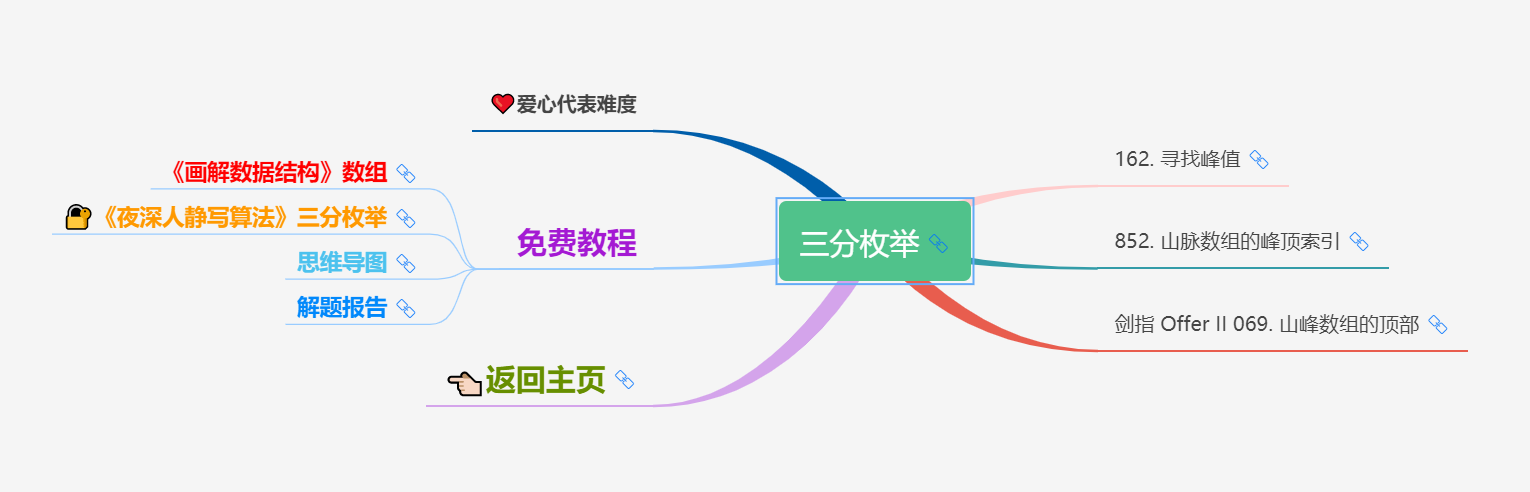### 4）前缀和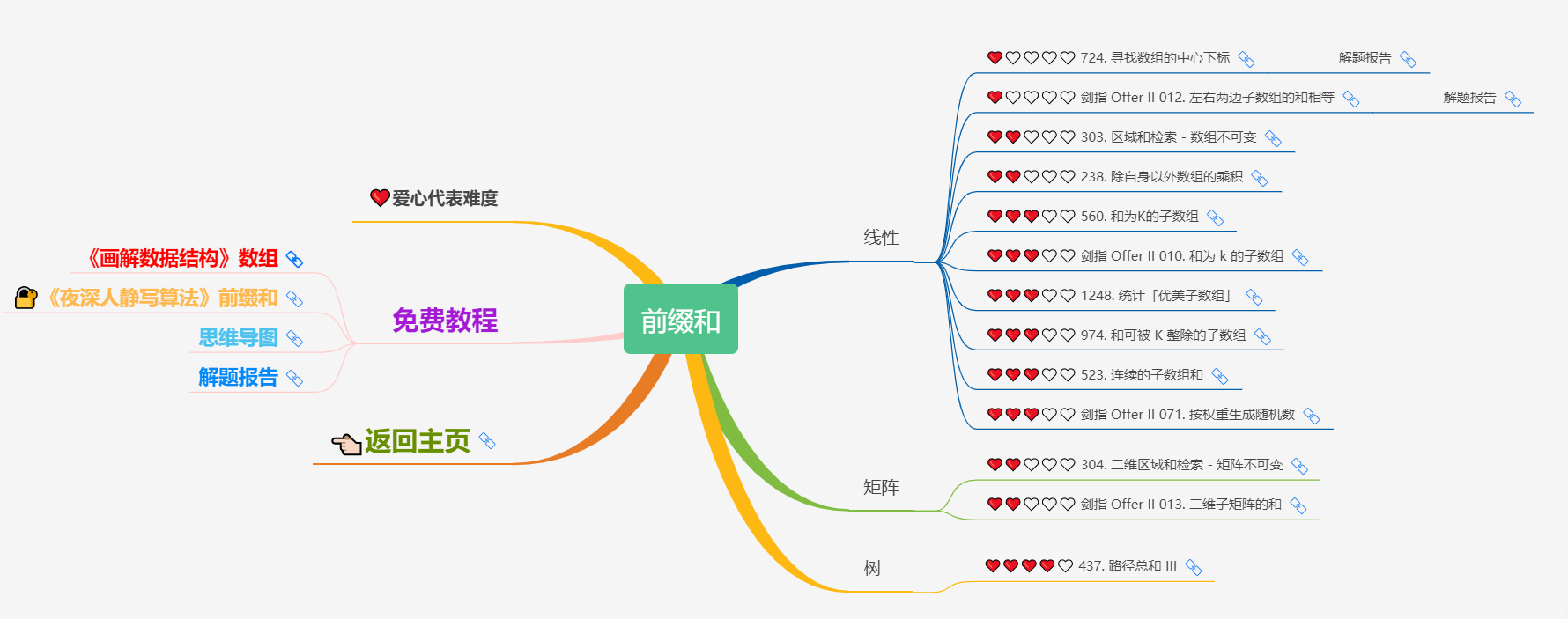### 5）双指针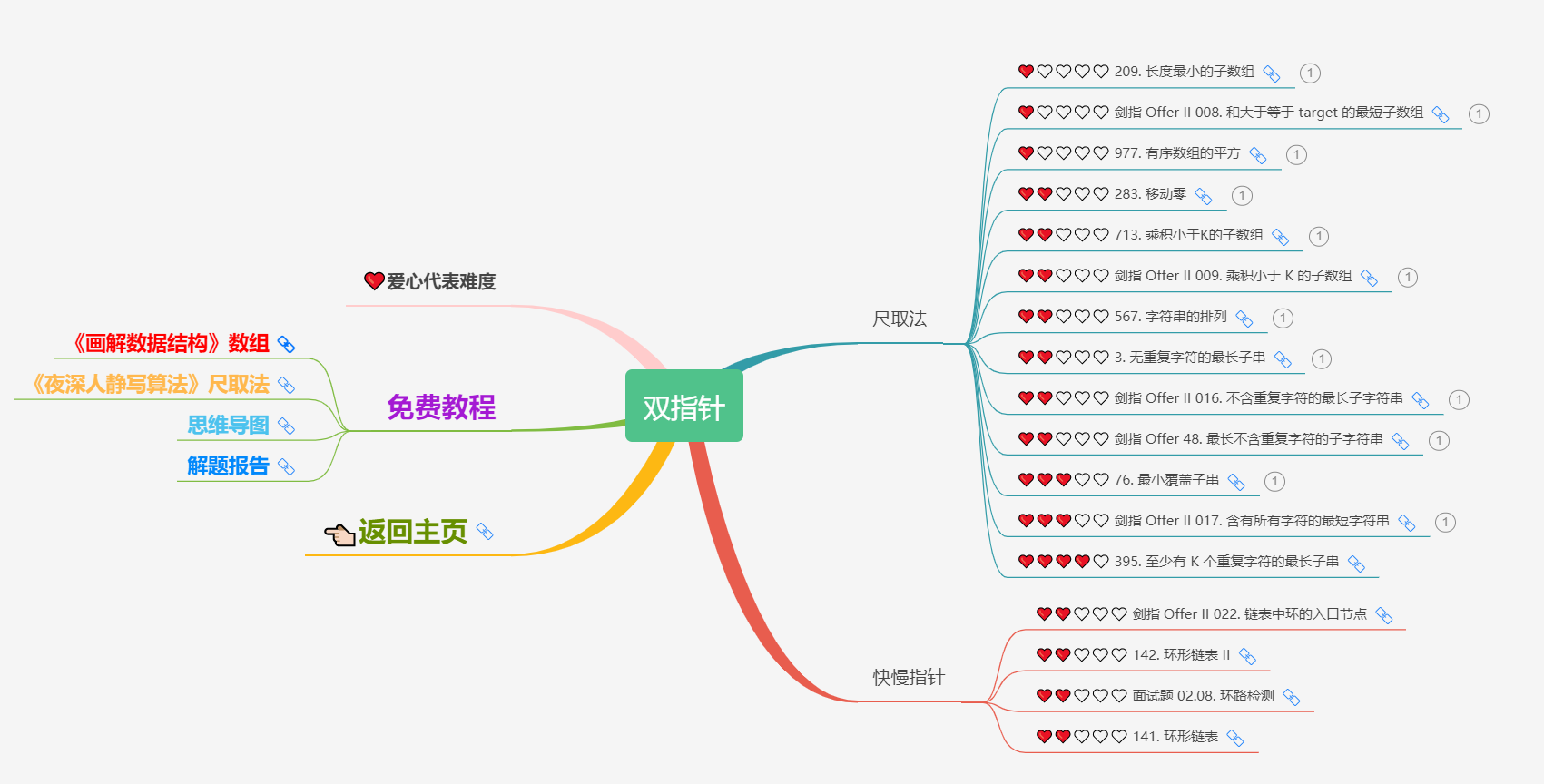给定一个长度为 n ( 1 ≤ n ≤ 1 0 7 ) n (1 \le n \le 10^7) 的字符串 s s ，求一个最长的满足所有字符不重复的子串的长度。
样例输入：" a b c a b c b b g abcabcbbg "
样例输出： 3 3
原题出处： LeetCode 3. 无重复字符的最长子串

我们考虑一个子串以 s i s_i 为左端点， s j s_j 为右端点，且 s [ i : j − 1 ] s[i:j-1] 中不存在重复字符， s [ i : j ] s[i:j] 中存在重复字符（换言之， s j s_j s [ i : j − 1 ] s[i:j-1] 中某个字符相同）。如图所示：那么我们没必要再去检测 s [ i : j + 1 ] s[i:j+1] s [ i : j + 2 ] s[i:j+2] s [ i : n − 1 ] s[i:n-1] 这几个字符串的合法性，因为当前情况 s [ i : j ] s[i:j] 是非法的，而这些字符串是完全包含 s [ i : j ] s[i:j] 的，所以它们必然也是不合法的。
那么我们可以把枚举的左端点自增，即： i = i + 1 i = i +1 ，这时，按照朴素算法的实现，右端点需要重置，即 j = i j = i ，实际上这里的右端点可以不动。
可以这么考虑，由于 s j s_j 这个字符和 s [ i : j − 1 ] s[i:j-1] 中的字符产生了重复，假设这个重复的字符的下标为 k k ，那么 i i 必须满足 i > k i \gt k ，换言之， i i 可以一直自增，直到 i = k + 1 i = k+1 ，如图所示：这个问题是 双指针 问题的原题，详细的算法可以参考：夜深人静写算法（二十八）- 尺取法
两个指针都只会递增各一次，所以时间复杂度为 O ( n ) O(n)

class Solution {

int hash;

public:
int lengthOfLongestSubstring(string s) {

memset(hash, 0, sizeof(hash));
int maxLen = 0;
int i = 0, j = -1;             // (1)
int len = s.length();          // (2)
while(j++ < len - 1) {

++hash[ s[j] ];            // (3)
while(hash[ s[j] ] > 1) {
// (4)
--hash[ s[i] ];        // (5)
++i;
}
if(j - i + 1 > maxLen) {
// (6)
maxLen = j - i + 1;
}
}
return maxLen;
}
};

• ( 1 ) (1) 代表一开始是空串；
• ( 2 ) (2) 之所以要这么做，是因为s.length()是无符号整型，当 j == -1的情况为无符号整型的最大值，永远都无法进入下面的while(j++ < len - 1)这个循环；
• ( 3 ) (3) 尝试向右移动 右指针；
• ( 4 ) (4) 合法性判定：所有字符个数不超过1；
• ( 5 ) (5) 尝试向右移动 左指针；
• ( 6 ) (6) 更新最大合法长度；

## 3、动态规划### 1）递推问题

递推问题作为动态规划的基础，是最好掌握的，也是必须掌握的，它有点类似于高中数学中的数列，通过 前几项的值 推导出 当前项的值

你正在爬楼梯，需要 n n 阶你才能到达楼顶。每次你可以爬 1 1 2 2 个台阶。你有多少种不同的方法可以爬到楼顶呢？假设我们已经到了第 n n 阶楼梯，那么它可以是从 n − 1 n-1 阶过来的，也可以是从 n − 2 n-2 阶过来的（但是，不可能是从 n − 3 n-3 阶直接过来的），所以如果达到第 n n 阶的方案数为 f [ n ] f[n] ，那么到达 n − 1 n-1 阶就是 f [ n − 1 ] f[n-1] ，到达 n − 2 n-2 阶 就是 f [ n − 2 ] f[n-2] ，所以可以得出： f [ n ] = f [ n − 1 ] + f [ n − 2 ] f[n] = f[n-1] + f[n-2]   其中，当 n = 0 n=0 时方案数为 1，代表初始情况； n = 1 n=1 时方案数为 1，代表走了一步，递推计算即可。
以上就是最简单的动态规划问题，也是一个经典的数列：斐波那契数列 的求解方式。它通过一个递推公式，将原本指数级的问题转化成了线性的，时间复杂度为 O ( n ) O(n)
C语言代码实现如下：

int f;
int climbStairs(int n){

f = f = 1;
for(int i = 2; i <= n; ++i) {

f[i] = f[i-1] + f[i-2];
}
return f[n];
}


### 2）线性DP

1、最小花费

数组的每个下标作为一个阶梯，第 i i 个阶梯对应着一个非负数的体力花费值 c o s t [ i ] cost[i] （下标从 0 开始）。每当爬上一个阶梯，都要花费对应的体力值，一旦支付了相应的体力值，就可以选择 向上爬一个阶梯 或者 爬两个阶梯。求找出达到楼层顶部的最低花费。在开始时，可以选择从下标为 0 0 1 1 的元素作为初始阶梯。令走到第 i i 层的最小消耗为 f [ i ] f[i] 。假设当前的位置在 i i 层楼梯，那么只可能从 i − 1 i-1 层过来，或者 i − 2 i-2 层过来；
如果从 i − 1 i-1 层过来，则需要消耗体力值： f [ i − 1 ] + c o s t [ i − 1 ] f[i-1] + cost[i-1]
如果从 i − 2 i-2 层过来，则需要消耗体力值： f [ i − 2 ] + c o s t [ i − 2 ] f[i-2] + cost[i-2]
起点可以在第 0 或者 第 1 层，于是有状态转移方程： f [ i ] = { 0 i = 0 , 1 min ⁡ ( f [ i − 1 ] + c o s t [ i − 1 ] , f [ i − 2 ] + c o s t [ i − 2 ] ) i > 1 f[i] = \begin{cases} 0 & i=0,1\\ \min ( f[i-1] + cost[i-1], f[i-2] + cost[i-2] ) & i > 1\end{cases}这个问题和一开始的递推问题的区别在于：一个是求前两项的和，一个是求最小值。这里就涉及到了动态取舍的问题，也就是动态规划的思想。
如果从前往后思考，每次都有两种选择，时间复杂度为 O ( 2 n ) O(2^n) 。转化成动态规划以后，只需要一个循环，时间复杂度为 O ( n ) O(n)
C语言代码实现如下：

int f;
int min(int a, int b) {

return a < b ? a : b;
}
int minCostClimbingStairs(int* cost, int costSize){

f = 0;
f = 0;
for(int i = 2; i <= costSize; ++i) {

f[i] = min(f[i-1] + cost[i-1], f[i-2] + cost[i-2]);
}
return f[costSize];
}


2、最大子段和

给定一个整数数组 n u m s nums ，找到一个具有最大和的连续子数组（子数组最少包含一个元素），返回其最大和。

由于要求的是连续的子数组，所以对于第 i i 个元素，状态转移一定是从 i − 1 i-1 个元素转移过来的。基于这一点，可以令 f [ i ] f[i] 表示以 i i 号元素结尾的最大值。
那么很自然，这个最大值必然包含 n u m s [ i ] nums[i] 这个元素，那么要不要包含 n u m s [ i − 1 ] , n u m s [ i − 2 ] , n u m s [ i − 3 ] , . . . , n u m s [ k ] nums[i-1],nums[i-2],nums[i-3],...,nums[k] 呢？其实就是看第 i − 1 i-1 号元素结尾的最大值是否大于零，也就是：当 f [ i − 1 ] ≤ 0 f[i-1] \le 0 时，则 前 i − 1 i-1 个元素是没必要包含进来的。所以就有状态转移方程： f [ i ] = { n u m s [ 0 ] i = 0 n u m s [ i ] f [ i − 1 ] ≤ 0 n u m s [ i ] + f [ i − 1 ] f [ i − 1 ] > 0 f[i] = \begin{cases} nums & i = 0 \\ nums[i] & f[i-1] \le 0 \\ nums[i] + f[i-1] & f[i-1] > 0\end{cases} 一层循环枚举后，取 m a x ( f [ i ] ) max(f[i]) 就是答案了。只需要一个循环，时间复杂度为 O ( n ) O(n)
C语言代码实现如下：

int dp;
int max(int a, int b) {

return a > b ? a : b;
}

int maxSubArray(int* nums, int numsSize){

int maxValue = nums;
dp = nums;
for(int i = 1; i < numsSize; ++i) {

dp[i] = nums[i];
if(dp[i-1] > 0) {

dp[i] += dp[i-1];
}
maxValue = max(maxValue, dp[i]);
}
return maxValue;
}


3、最长单调子序列

给定一个长度为 n ( 1 ≤ n ≤ 1000 ) n(1 \le n \le 1000) 的数组 a i a_i ，求给出它的最长递增子序列的长度。

在看这个问题之前，我们先来明确一些概念：单调序列、单调子序列、最大长单调子序列。
单调序列 就是一个满足某种单调性的数组序列，比如 单调递增序列、单调递减序列、单调不增序列、单调不减序列。举几个简单的例子：
单调递增序列：1，2，3，7，9
单调递减序列：9，8，4，2，1
单调不增序列：9，8，8，5，2
单调不减序列：1，2，2，5，5
一个比较直观的单调递增序列的例子就是一个楼梯的侧面。我们可以把这个楼梯每一阶的高度用一个数字表示，得到一个单调递增序列，如图所示：单调子序列 指的是任意一个数组序列，按顺序选择一些元素组成一个新的序列，并且满足单调性。对于一个长度为 n n 的序列，每个元素可以选择 “取” 或者 “不取”，所以最多情况下，有 2 n 2^n 个单调子序列。
如图所示，代表的是序列：[1，2，4，6，3，5，9]其中 [1，2，6] 为它的一个长度为 3 的单调子序列，如图所示；[1，3，6] 则不是，因为 3 和 6 的顺序不是原序列中的顺序；[1，4，3] 也不是，因为它不满足单调性。
最长单调子序列 是指对于原数组序列，能够找到的元素个数最多的单调子序列。
还是以 [1，2，4，6，3，5，9] 为例，它的最长单调子序列为：[1，2，4，6，9]，长度为 5；当然，也可以是 [1，2，3，5，9]，长度同样为 5。那么，接下来，我们看下如何通过动态规划的方法来求解 最长递增子序列
对于数组序列 a i ( 1 ≤ i ≤ n ) a_i(1 \le i \le n) ，令 f [ i ] f[i] 表示以第 i i 个数 a i a_i 结尾的最长递增子序列的长度。那么，我们考虑以第 i i 个数 a i a_i 结尾的最长递增子序列，它在这个序列中的前一个数一定是 a j ( 1 ≤ j < i ) a_j(1 \le j < i) 中的一个，所以，如果我们已经知道了 f [ j ] f[j] ，那么就有 f [ i ] = f [ j ] + 1 f[i] = f[j] + 1 。显然，我们还需要满足 a j < a i a_j < a_i 这个递增的限制条件。
那么就可以得出状态转移方程： f [ i ] = max ⁡ j = 1 i − 1 ( f [ j ]   ∣   a j < a i ) + 1 f[i] = \max_{j=1}^{i-1} (f[j] \ | \ a_j < a_i) + 1   这里 f [ j ] f[j] f [ i ] f[i] 的子结构，而 m a x ( f [ j ] ) max(f[j]) f [ i ] f[i] 的最优子结构，当然我们需要考虑一种情况，就是没有找到最优子结构的时候，例如： i = 1 i=1 或者 不存在 a j < a i a_j < a_i j j ，此时 f [ i ] = 1 f[i] = 1 ，表示 a i a_i 本身是一个长度为 1 1 的最长递增子序列。
f [ i ] f[i] 数组可以通过两层循环来求解，如下图表所示：状态数 f [ . . . ] f[...] 总共 O ( n ) O(n) 个，状态转移的消耗为 O ( n ) O(n) ，所以总的时间复杂度为 O ( n 2 ) O(n^2) ，所以对于这类问题，一般能够接受的 n n 的范围在千级别，也就是 1000 , 2000 , 3000... 1000, 2000, 3000 ... 。如果是 n = 10000 , 100000 n=10000, 100000 的情况，就需要考虑优化了。
有关最长单调子序列的问题，还有 O ( n l o g 2 n ) O(nlog_2n) 的优化算法，具体方法可以参考以下文章：夜深人静写算法（二十）- 最长单调子序列

### 3）二维DP

1、最长公共子序列

给定两个数组序列 a 1 , a 2 , . . . , a n a_1, a_2, ..., a_n b 1 , b 2 , . . . , b m b_1, b_2, ..., b_m ，其中 n , m ≤ 1000 n,m \le 1000 ，求两个数组的最长公共子序列。

考虑两个数组序列 a 1 , a 2 , . . . , a n a_1, a_2, ..., a_n b 1 , b 2 , . . . , b m b_1, b_2, ..., b_m ，对于 a a 序列中第 i i 个元素 a i a_i b b 序列中的第 j j 个元素 b j b_j ，有两种情况：
( 1 ) (1) 相等即 a i = = b j a_i == b_j 的情况，这个时候如果前缀序列 a 1 , a 2 , . . . , a i − 1 a_1, a_2, ..., a_{i-1} b 1 , b 2 , . . . , b j − 1 b_1, b_2, ..., b_{j-1} 的最长公共子序列已经求出来了，记为 x x 的话，那么很显然，在两个序列分别加入 a i a_i b j b_j 以后，长度又贡献了 1，所以 a 1 , a 2 , . . . , a i a_1, a_2, ..., a_{i} b 1 , b 2 , . . . , b j b_1, b_2, ..., b_{j} 的最长公共子序列就是 x + 1 x+1( 2 ) (2) 不相等即 a i ≠ b j a_i \neq b_j 的情况，这个时候我们可以把问题拆分成两个更小的子问题，即 分别去掉 a i a_i b j b_j 的情况，如图所示：去掉 a i a_i 以后，问题转变为求： a 1 , a 2 , . . . , a i − 1 a_1, a_2, ..., a_{i-1} b 1 , b 2 , . . . , b j b_1, b_2, ..., b_{j} 的最长公共子序列；
去掉 b j b_j 以后，问题转变为求： a 1 , a 2 , . . . , a i a_1, a_2, ..., a_{i} b 1 , b 2 , . . . , b j − 1 b_1, b_2, ..., b_{j-1} 的最长公共子序列；
根据上面的两种情况的讨论，我们发现，在任何时候，我们都在求 a a 的前缀 和 b b 的前缀的最长公共序列，所以可以这么定义状态：用 f [ i ] [ j ] f[i][j] 表示 a 1 , a 2 , . . . , a i a_1, a_2, ..., a_{i} b 1 , b 2 , . . . , b j b_1, b_2, ..., b_{j} 的最长公共子序列。
在设计状态的过程中，我们已经无形中把状态转移也设计好了，状态转移方程如下： f [ i ] [ j ] = { 0 i = 0   o r   j = 0 f [ i − 1 ] [ j − 1 ] + 1 i , j > 0 , a i = b j max ⁡ ( f [ i ] [ j − 1 ] , f [ i − 1 ] [ j ] ) i , j > 0 , a i ≠ b j f[i][j] = \begin{cases}0 & i=0\ or\ j=0 \\ f[i-1][j-1] + 1 & i,j>0,a_i=b_j \\ \max(f[i][j-1], f[i-1][j]) & i,j>0,a_i \neq b_j\end{cases}   对于 i = 0 i=0 或者 j = 0 j=0 代表的是：其中一个序列的长度为 0，那么最长公共子序列的长度肯定就是 0 了；
其余两种情况，就是我们上文提到的 a i a_i b j b_j “相等” 与 “不相等” 的两种情况下的状态转移。如图所示，代表了字符串 “GATCGTGAGC” 和 “AGTACG” 求解最长公共子序列的 f [ i ] [ j ] ( i , j > 0 ) f[i][j] (i,j > 0) 的矩阵。对于长度分别为 n n m m 的两个序列，求解它们的最长公共子序列时，状态数总共有 O ( n m ) O(nm) 个，每次状态转移的消耗为 O ( 1 ) O(1) ，所以总的时间复杂度为 O ( n m ) O(nm)
对于 f [ i ] [ j ] f[i][j] 这个状态，求解过程中，只依赖于 f [ i ] [ j − 1 ] f[i][j-1] f [ i − 1 ] [ j − 1 ] f[i-1][j-1] f [ i − 1 ] [ j ] f[i-1][j]即每次求解只需要有 上一行 和 这一行 的状态即可，所以可以采用滚动数组进行优化，将状态转移方程变成： f [ c u r ] [ j ] = { f [ l a s t ] [ j − 1 ] + 1 j > 0 , a i = b j max ⁡ ( f [ c u r ] [ j − 1 ] , f [ l a s t ] [ j ] ) j > 0 , a i ≠ b j f[cur][j] = \begin{cases}f[last][j-1] + 1 & j>0,a_i=b_j \\ \max(f[cur][j-1], f[last][j]) & j>0,a_i \neq b_j\end{cases}   只需要简单将 i i 替换成 c u r cur i − 1 i-1 替换成 l a s t last 即可。这样就把原本 O ( n m ) O(nm) 的空间复杂度变成了 O ( p ) O(p) ，其中 p = min ⁡ ( n , m ) p = \min(n,m)

• 优化后的 C++ 代码实现如下：
typedef char ValueType;
const int maxn = 5010;
int f[maxn];

int getLCSLength(int hsize, ValueType *h, int vsize, ValueType *v) {

memset(f, 0, sizeof(f));
int cur = 1, last = 0;
for (int i = 1; i <= vsize; ++i) {

for (int j = 1; j <= hsize; ++j) {

if (v[i] == h[j])
f[cur][j] = f[last][j - 1] + 1;
else
f[cur][j] = max(f[cur][j - 1], f[last][j]);
}
swap(last, cur);
}
return f[last][hsize];
}


有关于 最长公共子序列 的更多内容，可以参考以下内容：夜深人静写算法（二十一）- 最长公共子序列

2、最小编辑距离

长度为 n n 的源字符串 a 1 , a 2 , . . . , a n a_1,a_2,...,a_n ，经过一些给定操作变成长度为 m m 的目标字符串 b 1 , b 2 , . . . b m b_1,b_2,...b_m ，操作包括如下三种：
1） I n s e r t Insert ：在源字符串中插入一个字符，插入消耗为 I I
2） D e l e t e Delete ：在源字符串中删除一个字符，删除消耗为 D D
3） R e p l a c e Replace ：将源字符串中的一个字符替换成另一个字符，替换消耗为 R R

令 f [ i ] [ j ] f[i][j] 表示源字符串 a 1 , a 2 , . . . , a i a_1,a_2,...,a_i 经过上述三种操作变成目标字符串 b 1 , b 2 , . . . b j b_1,b_2,...b_j 的最少消耗。
假设 a 1 , a 2 , . . . , a i a_1,a_2,...,a_{i} 变成 b 1 , b 2 , . . . b j − 1 b_1,b_2,...b_{j-1} 的最少消耗已经求出，等于 f [ i ] [ j − 1 ] f[i][j-1] ，则需要在 a [ i ] a[i] 的后面插入一个字符 b j b_j ，那么产生的消耗为： f [ i ] [ j − 1 ] + I f[i][j-1] + I 如图所示，源字符串为 “AGTA”，目标字符串为 “GATCGT” 的情况下，将源字符串变成 "“GATCG” 的最小消耗为 f [ i ] [ j − 1 ] f[i][j-1] ，那么只要在源字符串最后再插入一个 ‘T’，就可以把源字符串变成目标字符串 “GATCGT”；假设 a 1 , a 2 , . . . , a i − 1 a_1,a_2,...,a_{i-1} 变成 b 1 , b 2 , . . . b j b_1,b_2,...b_{j} 的最少消耗已经求出，等于 f [ i − 1 ] [ j ] f[i-1][j] ，则需要把 a i a_i 个删掉，那么产生的消耗为： f [ i − 1 ] [ j ] + D f[i-1][j] + D 如图所示，源字符串为 “AGTA”，目标字符串为 “GATCGT” 的情况下，将 “AGT” 变成目标字符串的最小消耗为 f [ i − 1 ] [ j ] f[i-1][j] ，那么只要把源字符串最后一个’A’删掉，就可以把源字符串变成目标字符串；假设 a 1 , a 2 , . . . , a i − 1 a_1,a_2,...,a_{i-1} 变成 b 1 , b 2 , . . . b j − 1 b_1,b_2,...b_{j-1} 的最少消耗已经求出，等于 f [ i − 1 ] [ j − 1 ] f[i-1][j-1] ，则将 a i a_i 替换成 b j b_j a 1 , a 2 , . . . , a i a_1,a_2,...,a_{i} 就可以变成 b 1 , b 2 , . . . b j b_1,b_2,...b_{j} 。替换时需要考虑 a i = b j a_i=b_j a i ≠ b j a_i \neq b_j 的情况，所以替换产生的消耗为： f [ i − 1 ] [ j − 1 ] + { 0 a i = b j R a i ≠ b j f[i-1][j-1] + \begin{cases} 0 & a_i=b_j \\ R & a_i \neq b_j\end{cases} 如图所示，源字符串为 “AGTA”，目标字符串为 “GATCGT” 的情况下，将 “AGT” 变成 “GATCGT” 的最小消耗为 f [ i − 1 ] [ j − 1 ] f[i-1][j-1] ，那么只要将 源字符串 的最后一个字符 替换为 目标字符串 的最后一个字符 ，就可以把源字符串变成目标字符串；替换时根据 源字符串 和 目标字符串 原本是否相等来决定消耗；• 边界情况主要考虑以下几种：
a. 空串变成目标串 f [ 0 ] [ j ] f[j] ，总共需要插入 j j 个字符，所以 f [ 0 ] [ j ] = f [ 0 ] [ j − 1 ] + I f[j] = f[j-1] + I
b. 源字符串变成空串 f [ i ] [ 0 ] f[i] ，总共需要删除 i i 个字符，所以 f [ i ] [ 0 ] = f [ i − 1 ] [ 0 ] + D f[i] = f[i-1] + D
c. 空串变成空串 f [ 0 ] [ 0 ] = 0 f = 0

将上述所有状态进行一个整合，得到状态转移方程如下： f [ i ] [ j ] = { 0 i = 0 , j = 0 f [ i ] [ j − 1 ] + I i = 0 , j > 0 f [ i − 1 ] [ j ] + D i > 0 , j = 0 min ⁡ i > 0 , j > 0 { f [ i ] [ j − 1 ] + I f [ i − 1 ] [ j ] + D f [ i − 1 ] [ j − 1 ] + R a i ≠ b j f[i][j] = \begin{cases}0 & i=0,j=0\\f[i][j-1]+I & i=0,j>0\\ f[i-1][j] + D & i>0,j=0 \\ \min_{i>0,j>0} \begin{cases} f[i][j-1] + I\\ f[i-1][j] + D\\ f[i-1][j-1] + R_{a_i \neq b_j}\end{cases}\end{cases}
通过这个状态矩阵，最后计算得到 f [ n ] [ m ] f[n][m] 就是该题所求 "源字符串 a 1 , a 2 , . . . , a n a_1,a_2,...,a_n ，经过 插入、删除、替换 变成目标字符串 b 1 , b 2 , . . . b m b_1,b_2,...b_m " 的最少消耗了，特殊的，当 I = D = R = 1 I = D = R = 1 时， f [ n ] [ m ] f[n][m] 就是字符串 a a b b 的莱文斯坦距离。
状态总数 O ( n m ) O(nm) ，每次状态转移的消耗为 O ( 1 ) O(1) ，所以总的时间复杂度为 O ( n m ) O(nm) ，空间上可以采用滚动数组进行优化，具体优化方案可以参考 最长公共子序列 的求解过程。
如图所示的是一张源字符串 “AGTACG” 到目标字符串 “GATCGTGAGC” 的莱文斯坦距离图。有关最小编辑距离的详细内容，可以参考：夜深人静写算法（二十二）- 最小编辑距离

3、双串匹配问题

给定一个 匹配字符串 s (只包含小写字母) 和一个 模式字符串 p (包含小写字母和两种额外字符：'.''*')，要求实现一个支持 '.''*'的正则表达式匹配（'*'前面保证有字符）。
'.'匹配任意单个字符
'*'匹配零个或多个前面的那一个元素

这是个经典的 串匹配 问题，可以按照 最长公共子序列 的思路去解决。令 f ( i , j ) f(i, j) 代表的是 匹配串前缀 s[0:i]模式串前缀 p[0:j] 是否有匹配，只有两个值： 0 代表 不匹配， 1 代表 匹配
于是，对模式串进行分情况讨论：
1）当 p[j].时，代表 s[i] 为任意字符时，它都能够匹配（没毛病吧？没毛病），所以问题就转化成了求 匹配串前缀 s[0:i-1]模式串前缀 p[0:j-1] 是否有匹配的问题，也就是这种情况下 f ( i , j ) = f ( i − 1 , j − 1 ) f(i, j) = f(i-1, j-1) ，如图1所示：2）当 p[j]*时，由于*前面保证有字符，所以拿到字符 p[j-1]，分情况讨论：
2.a）如果 p[j-1].时，可以匹配所有 s[0:i] 的后缀，这种情况下，只要 f ( k , j − 2 ) f(k, j-2) 为 1， f ( i , j ) f(i, j) 就为 1；其中 k ∈ [ 0 , i ] k \in [0, i] 。如下图所示：2.b）如果 p[j-1].时，只有当 s[0:i] 的后缀 字符全为 p[j-1] 时，才能去匹配 s[0:i] 的前缀，同样转化成 f ( k , j − 2 ) f(k, j-2) 的子问题。如下图所示：3）当 p[j] 为其他任意字符时，一旦 p[j]s[i] 不匹配，就认为 f ( i , j ) = 0 f(i, j) = 0 ，否则 f ( i , j ) = f ( i − 1 , j − 1 ) f(i, j) = f(i-1, j-1) ，如下图所示：最后，这个问题可以采用 记忆化搜索 求解，并且需要考虑一些边界条件，边界条件可以参考代码实现中的讲解。记忆化搜索会在下文仔细讲解。
匹配串的长度为 n n ，模式串的长度为 m m 。状态数： O ( n m ) O(nm) ，状态转移： O ( n ) O(n) ，时间复杂度： O ( n 2 m ) O(n^2m)

### 4）记忆化搜索

给定一个 n ( n ≤ 45 ) n(n \le 45) ，求 斐波那契数列的第 n n 项的值，要求用递归实现。

那么，我们只需要套用上面的递归函数，并且处理好递归出口，就能把它写成递归的形式，C语言 代码实现如下：

int f(unsigned int n) {

if(n <= 1) {

return 1;
}
return f(n-1) + f(n-2);
}


递归求解的过程如下：这是一棵二叉树，树的高度为 n n ，所以粗看递归访问时结点数为 2 n 2^n ，但是仔细看，对于任何一棵子树而言，左子树的高度一定比右子树的高度大，所以不是一棵严格的完全二叉树。为了探究它实际的时间复杂度，我们改下代码：

int f(unsigned int n) {

++c[n];
if(n <= 1) {

return 1;
}
return f(n-1) + f(n-2);
}


加了一句代码 ++c[n];，引入一个计数器，来看下在不同的 n n 的情况下， f ( n ) f(n) 这个函数的调用次数，如图所示：观察 c [ n ] c[n] 的增长趋势，首先排除等差数列，然后再来看是否符合等比数列，我们来尝试求下 c [ n ] / c [ n − 1 ] c[n] / c[n-1] 的值，列出表格如下：观察发现，随着 n n 的不断增大， c [ n ] / c [ n − 1 ] c[n]/c[n-1] 越来越接近一个常数，而这个常数就是黄金分割的倒数： 2 5 − 1 ≈ 1.618034 \frac {2}{ \sqrt 5 - 1} \approx 1.618034   当 n n 趋近于无穷大的时候，满足如下公式： c [ n ] = 2 5 − 1 c [ n − 1 ] c[n] = \frac {2}{ \sqrt 5 - 1} c[n-1]   对等比数列化解后累乘得到： c [ n ] = 2 5 − 1 c [ n − 1 ] = ( 2 5 − 1 ) 2 c [ n − 2 ] = ( 2 5 − 1 ) n \begin{aligned}c[n] &= \frac {2}{ \sqrt 5 - 1} c[n-1]\\ &= (\frac {2}{ \sqrt 5 - 1})^2 c[n-2]\\ &= (\frac {2}{ \sqrt 5 - 1})^n \end{aligned}   所以，斐波那契数列递归求解的时间复杂度就是 ： O ( ( 2 5 − 1 ) n ) O((\frac {2}{ \sqrt 5 - 1})^n)
有关记忆化搜索的更多内容，可以参考：夜深人静写算法（二十六）- 记忆化搜索

### 5）背包问题

1、0/1 背包

有 n ( n ≤ 100 ) n(n \le100) 个物品和一个容量为 m ( m ≤ 10000 ) m(m \le 10000) 的背包。第 i i 个物品的容量是 c [ i ] c[i] ，价值是 w [ i ] w[i] 。现在需要选择一些物品放入背包，并且总容量不能超过背包容量，求能够达到的物品的最大总价值。

以上就是 0/1 背包问题的完整描述，之所以叫 0/1 背包，是因为每种物品只有一个，可以选择放入背包或者不放，而 0 代表不放，1 代表放。
第一步：设计状态；
状态 ( i , j ) (i, j) 表示前 i i 个物品恰好放入容量为 j j 的背包 ( i ∈ [ 0 , n ] , j ∈ [ 0 , m ] ) (i \in [0, n], j \in [0, m])
令 d p [ i ] [ j ] dp[i][j] 表示状态 ( i , j ) (i, j) 下该背包得到的最大价值，即前 i i 个物品恰好放入容量为 j j 的背包所得到的最大总价值；
第二步：列出状态转移方程； d p [ i ] [ j ] = m a x ( d p [ i − 1 ] [ j ] , d p [ i − 1 ] [ j − c [ i ] ] + w [ i ] ) dp[i][j] = max(dp[i-1][j], dp[i-1][j - c[i]] + w[i]) 因为每个物品要么放，要么不放，所以只需要考虑第 i i 个物品 放 或 不放 的情况：
1）不放：如果 “第 i i 个物品不放入容量为 j j 的背包”，那么问题转化成求 “前 i − 1 i-1 个物品放入容量为 j j 的背包” 的问题；由于不放，所以最大价值就等于 “前 i − 1 i-1 个物品放入容量为 j j 的背包” 的最大价值，即 d p [ i − 1 ] [ j ] dp[i-1][j]
2）放：如果 “第 i i 个物品放入容量为 j j 的背包”，那么问题转化成求 “前 i − 1 i-1 个物品放入容量为 j − c [ i ] j-c[i] 的背包” 的问题；那么此时最大价值就等于 “前 i − 1 i-1 个物品放入容量为 j − c [ i ] j-c[i] 的背包” 的最大价值 加上放入第 i i 个物品的价值，即 d p [ i − 1 ] [ j − c [ i ] ] + w [ i ] dp[i-1][j - c[i]] + w[i]
将以上两种情况取大者，就是我们所求的 “前 i i 个物品恰好放入容量为 j j 的背包” 的最大价值了。我们发现，当状态在进行转移的时候， ( i , j ) (i, j) 不是来自 ( i − 1 , j ) (i-1, j) ，就是来自 ( i − 1 , j − c [ i ] ) (i-1, j - c[i]) ，所以必然有一个初始状态，而这个初始状态就是 ( 0 , 0 ) (0, 0) ，含义是 “前 0 个物品放入一个背包容量为 0 的背包”，这个状态下的最大价值为 0，即 d p [ 0 ] [ 0 ] = 0 dp = 0
那么我们再来考虑， ( 0 , 3 ) (0, 3) 是什么意思呢？它代表的是 “前 0 个物品恰好放入一个背包容量为 3 的背包”，明显这种情况是不存在的，因为 0 个物品的价值肯定是 0。所以这种状态被称为非法状态，非法状态是无法进行状态转移的，于是我们可以通过初始状态和非法状态进所有状态进行初始化。
d p [ 0 ] [ i ] = { 0 i = 0 i n f i > 0 dp[i] = \begin{cases} 0 & i = 0\\ inf & i > 0\end{cases}
其中 i n f inf 在程序实现时，我们可以设定一个非常小的数，比如 − 1000000000 -1000000000 ，只要保证无论如何状态转移它都不能成为最优解的候选状态。为了加深状态转移的概念，来看图二-5-1 的一个例子，每个格子代表一个状态， ( 0 , 0 ) (0,0) 代表初始状态，蓝色的格子代表已经求得的状态，灰色的格子代表非法状态，红色的格子代表当前正在进行转移的状态，图中的第 i i 行代表了前 i i 个物品对应容量的最优值，第 4 个物品的容量为 2，价值为 8，则有状态转移如下： d p [ 4 ] [ 4 ] = m a x ( d p [ 4 − 1 ] [ 4 ] , d p [ 4 − 1 ] [ 4 − 2 ] + 8 ) = m a x ( d p [ 3 ] [ 4 ] , d p [ 3 ] [ 2 ] + 8 ) \begin{aligned} dp &= max( dp[4-1], dp[4-1][4 - 2] + 8) \\ &= max( dp, dp + 8) \end{aligned}有关 0/1 背包的更多内容，可以参考：夜深人静写算法（十四）- 0/1 背包

2、完全背包

有 n ( n ≤ 100 ) n(n \le 100) 种物品和一个容量为 m ( m ≤ 10000 ) m(m \le 10000) 的背包。第 i i 种物品的容量是 c [ i ] c[i] ，价值是 w [ i ] w[i] 。现在需要选择一些物品放入背包，每种物品可以无限选择，并且总容量不能超过背包容量，求能够达到的物品的最大总价值。

以上就是完全背包问题的完整描述，和 0/1 背包的区别就是每种物品可以无限选取，即文中红色字体标注的内容；
第一步：设计状态；
状态 ( i , j ) (i, j) 表示前 i i 种物品恰好放入容量为 j j 的背包 ( i ∈ [ 0 , n ] , j ∈ [ 0 , m ] ) (i \in [0, n], j \in [0, m])
令 d p [ i ] [ j ] dp[i][j] 表示状态 ( i , j ) (i, j) 下该背包得到的最大价值，即前 i i 种物品（每种物品可以选择无限件）恰好放入容量为 j j 的背包所得到的最大总价值；

第二步：列出状态转移方程； d p [ i ] [ j ] = m a x ( d p [ i − 1 ] [ j − c [ i ] ∗ k ] + w [ i ] ∗ k ) dp[i][j] = max(dp[i-1][j - c[i]*k] + w[i]*k) ( 0 ≤ k ≤ j c [ i ] ) (0 \le k \le \frac {j} {c[i]})

• 因为每种物品有无限种可放置，将它归类为以下两种情况：
1）不放：如果 “第 i i 种物品不放入容量为 j j 的背包”，那么问题转化成求 “前 i − 1 i-1 种物品放入容量为 j j 的背包” 的问题；由于不放，所以最大价值就等于 “前 i − 1 i-1 种物品放入容量为 j j 的背包” 的最大价值，对应状态转移方程中 k = 0 k = 0 的情况， 即 d p [ i − 1 ] [ j ] dp[i-1][j]
2）放 k 个：如果 “第 i i 种物品放入容量为 j j 的背包”，那么问题转化成求 “前 i − 1 i-1 种物品放入容量为 j − c [ i ] ∗ k j-c[i]*k 的背包” 的问题；那么此时最大价值就等于 “前 i − 1 i-1 种物品放入容量为 j − c [ i ] ∗ k j-c[i]*k 的背包” 的最大价值 加上放入 k k 个第 i i 种物品的价值，即 d p [ i − 1 ] [ j − c [ i ] ∗ k ] + w [ i ] ∗ k dp[i-1][j - c[i]*k] + w[i]*k
枚举所有满足条件的 k k 就是我们所求的 “前 i i 种物品恰好放入容量为 j j 的背包” 的最大价值了。注意：由于每件物品都可以无限选择，所以这里描述的时候都是用的 “种” 作为单位，即代表不同种类的物品。

对于 n n 种物品放入一个容量为 m m 的背包，状态数为 O ( n m ) O(nm) ，每次状态转移的消耗为 O ( k ) O(k) ，所以整个状态转移的过程时间复杂度是大于 O ( n m ) O(nm) 的，那么实际是多少呢？考虑最坏情况下，即 c [ i ] = 1 c[i] = 1 时，那么要计算的 d p [ i ] [ j ] dp[i][j] 的转移数为 j j ，总的状态转移次数就是 m ( m + 1 ) 2 \frac {m(m + 1)} {2} ，所以整个算法的时间复杂度是 O ( n m 2 ) O(nm^2) 的，也就是说状态转移均摊时间复杂度是 O ( m ) O(m) 的。

我们把状态转移方程进行展开后得到如下的 k + 1 k+1 个式子：
d p [ i ] [ j ] = m a x { d p [ i − 1 ] [ j ] ( 1 ) d p [ i − 1 ] [ j − c [ i ] ] + w [ i ] ( 2 ) d p [ i − 1 ] [ j − c [ i ] ∗ 2 ] + w [ i ] ∗ 2 ( 3 ) . . . d p [ i − 1 ] [ j − c [ i ] ∗ k ] + w [ i ] ∗ k ( k + 1 ) dp[i][j] = max \begin{cases} dp[i-1][j] & (1)\\ dp[i-1][j - c[i]] + w[i] & (2)\\ dp[i-1][j - c[i]*2] + w[i]*2 & (3)\\ ... \\ dp[i-1][j - c[i]*k] + w[i] *k & (k+1) \end{cases}
利用待定系数法，用 j − c [ i ] j-c[i] 代替上式的 j j 得到如下式子：
d p [ i ] [ j − c [ i ] ] = m a x { d p [ i − 1 ] [ j − c [ i ] ] ( 1 ) d p [ i − 1 ] [ j − c [ i ] ∗ 2 ] + w [ i ] ( 2 ) d p [ i − 1 ] [ j − c [ i ] ∗ 3 ] + w [ i ] ∗ 2 ( 3 ) . . . d p [ i − 1 ] [ j − c [ i ] ∗ k ] + w [ i ] ∗ ( k − 1 ) ( k ) dp[i][j-c[i]] = max \begin{cases} dp[i-1][j-c[i]] & (1)\\ dp[i-1][j - c[i]*2] + w[i] & (2)\\ dp[i-1][j - c[i]*3] + w[i]*2 & (3)\\ ... \\ dp[i-1][j - c[i]*k] + w[i] *(k-1) & (k) \end{cases}
等式两边都加上 w [ i ] w[i] 得到：
d p [ i ] [ j − c [ i ] ] + w [ i ] = m a x { d p [ i − 1 ] [ j − c [ i ] ] + w [ i ] ( 1 ) d p [ i − 1 ] [ j − c [ i ] ∗ 2 ] + w [ i ] ∗ 2 ( 2 ) d p [ i − 1 ] [ j − c [ i ] ∗ 3 ] + w [ i ] ∗ 3 ( 3 ) . . . d p [ i − 1 ] [ j − c [ i ] ∗ k ] + w [ i ] ∗ k ( k ) dp[i][j-c[i]] + w[i] = max \begin{cases} dp[i-1][j-c[i]] + w[i] & (1)\\ dp[i-1][j - c[i]*2] + w[i]*2 & (2)\\ dp[i-1][j - c[i]*3] + w[i]*3 & (3)\\ ... \\ dp[i-1][j - c[i]*k] + w[i] *k & (k) \end{cases}
于是我们发现，这里的 ( 1 ) . . . ( k ) (1)...(k) 式子等价于最开始的状态转移方程中的 ( 2 ) . . . ( k + 1 ) (2) ... (k+1) 式，所以原状态转移方程可以简化为： d p [ i ] [ j ] = m a x ( d p [ i − 1 ] [ j ] , d p [ i ] [ j − c [ i ] ] + w [ i ] ) dp[i][j] = max(dp[i-1][j], dp[i][j-c[i]] + w[i])
这样就把原本均摊时间复杂度为 O ( m ) O(m) 的状态转移优化到了 O ( 1 ) O(1)
那么我们来理解一下这个状态转移方程的含义：对于第 i i 种物品，其实只有两种选择：一个都不放、至少放一个；一个都不放 就是 “前 i − 1 i-1 种物品放满一个容量为 j j 的背包” 的情况，即 d p [ i − 1 ] [ j ] dp[i-1][j] ；至少放一个 的话，我们尝试在 “前 i i 种物品放满一个容量为 j j 的背包” 里拿掉 1 个物品，即 “前 i i 种物品放满一个容量为 j − c [ i ] j-c[i] 的背包”，这时候的值就是 d p [ i ] [ j − c [ i ] ] + w [ i ] dp[i][j-c[i]] + w[i] 。取两者的大者就是答案了。
其实这个思路我可以在本文开头就讲，也容易理解，之所以引入优化以及逐步推导的过程，就是想告诉读者，很多动态规划的问题是不能套用模板的，从简单的思路出发，加上一些推导和优化，逐步把复杂的问题循序渐进的求出来，才是解决问题的普遍思路。
有关完全背包的更多内容，可以参考：夜深人静写算法（十五）- 完全背包

3、多重背包

有 n ( n ≤ 100 ) n(n \le 100) 种物品和一个容量为 m ( m ≤ 10000 ) m(m \le 10000) 的背包。第 i i 种物品的容量是 c [ i ] c[i] ，价值是 w [ i ] w[i] 。现在需要选择一些物品放入背包，每种物品可以选择 x [ i ] x[i] ，并且总容量不能超过背包容量，求能够达到的物品的最大总价值。

以上就是多重背包问题的完整描述，和 0/1 背包、完全背包的区别就是每种物品的选取有物品自己的值域限制，即文中红色字体标注的内容；
第一步：设计状态；
状态 ( i , j ) (i, j) 表示前 i i 种物品恰好放入容量为 j j 的背包 ( i ∈ [ 0 , n ] , j ∈ [ 0 , m ] ) (i \in [0, n], j \in [0, m])
令 d p [ i ] [ j ] dp[i][j] 表示状态 ( i , j ) (i, j) 下该背包得到的最大价值，即前 i i 种物品（每种物品可以选择 x [ i ] x[i] 件）恰好放入容量为 j j 的背包所得到的最大总价值；

第二步：列出状态转移方程； d p [ i ] [ j ] = m a x ( d p [ i − 1 ] [ j − c [ i ] ∗ k ] + w [ i ] ∗ k ) ( 0 ≤ k ≤ x [ i ] ) dp[i][j] = max(dp[i-1][j - c[i]*k] + w[i]*k) \\ (0 \le k \le x[i]) 因为每种物品有 x [ i ] x[i] 种可放置，将它归类为以下两种情况：
1）不放：如果 “第 i i 种物品不放入容量为 j j 的背包”，那么问题转化成求 “前 i − 1 i-1 种物品放入容量为 j j 的背包” 的问题；由于不放，所以最大价值就等于 “前 i − 1 i-1 种物品放入容量为 j j 的背包” 的最大价值，对应状态转移方程中 k = 0 k = 0 的情况， 即 d p [ i − 1 ] [ j ] dp[i-1][j]
2）放 k 个：如果 “第 i i 种物品放入容量为 j j 的背包”，那么问题转化成求 “前 i − 1 i-1 种物品放入容量为 j − c [ i ] ∗ k j-c[i]*k 的背包” 的问题；那么此时最大价值就等于 “前 i − 1 i-1 种物品放入容量为 j − c [ i ] ∗ k j-c[i]*k 的背包” 的最大价值 加上放入 k k 个第 i i 种物品的价值，即 d p [ i − 1 ] [ j − c [ i ] ∗ k ] + w [ i ] ∗ k dp[i-1][j - c[i]*k] + w[i]*k
枚举所有满足条件的 k k 就是我们所求的 “前 i i 种物品恰好放入容量为 j j 的背包” 的最大价值了。
多重背包问题是背包问题的一般情况，每种物品有自己的值域限制。如果从状态转移方程出发，我们可以把三种背包问题进行归纳统一，得到一个统一的状态转移方程如下： d p [ i ] [ j ] = m a x ( d p [ i − 1 ] [ j − c [ i ] ∗ k ] + w [ i ] ∗ k ) dp[i][j] = max(dp[i-1][j - c[i]*k] + w[i]*k)   对于 0/1 背包问题， k k 的取值为 0 , 1 0,1
对于完全背包问题， k k 的取值为 0 , 1 , 2 , 3 , . . . , ⌊ j c [ i ] ⌋ 0, 1, 2, 3, ..., \lfloor \frac j {c[i]} \rfloor
对于多重背包问题， k k 的取值为 0 , 1 , 2 , 3 , . . . , x [ i ] 0, 1, 2, 3, ..., x[i]
对于 n n 种物品放入一个容量为 m m 的背包，状态数为 O ( n m ) O(nm) ，每次状态转移的消耗为 O ( x [ i ] ) O(x[i]) ，所以整个状态转移的过程时间复杂度是大于 O ( n m ) O(nm) 的，那么实际是多少呢？
考虑最坏情况下，即 x [ i ] = m x[i] = m 时，那么要计算的 d p [ i ] [ j ] dp[i][j] 的转移数为 j j ，总的状态转移次数就是 m ( m + 1 ) 2 \frac {m(m + 1)} {2} ，所以整个算法的时间复杂度是 O ( n m 2 ) O(nm^2) 的，也就是说状态转移均摊时间复杂度是 O ( m ) O(m) 的。
一个容易想到的优化是：我们可以将每种物品拆成 x [ i ] x[i] 个，这样变成了 ∑ i = 1 n x [ i ] \sum_{i=1}^n x[i] 个物品的 0/1 背包问题，我们知道 0/1 背包问题优化完以后，空间复杂度只和容量有关，即 O ( m ) O(m)
所以多重背包问题的空间复杂度至少是可以优化到 O ( m ) O(m) 的。
然而， 如果这样拆分，时间复杂度还是没有变化，但是给我们提供了一个思路，就是每种物品是可以拆分的。假设有 x [ i ] x[i] 个物品，我们可以按照 2 的幂进行拆分，把它拆分成： 1 , 2 , 4 , . . . , 2 k − 1 , x [ i ] − 2 k + 1 1, 2, 4, ..., 2^{k-1}, x[i] - 2^{k} + 1
其中 k k 是最大的满足 x [ i ] − 2 k + 1 ≥ 0 x[i] - 2^{k} + 1 \ge 0 的非负整数。
这样，1 到 x [ i ] x[i] 之间的所有整数都能通过以上 k + 1 k+1 个数字组合出来，所以只要拆成以上 k + 1 k+1 个物品，所有取 k ( 0 ≤ k ≤ x [ i ] ) k (0 \le k \le x[i]) 个物品的情况都能被考虑进来。
举例说明，当 x [ i ] = 14 x[i] = 14 时，可以拆分成 1,2,4,7 个物品，那么当我们要取 13 个这类物品的时候，相当于选择 2、4、7，容量分别为 c [ i ] ∗ 2 , c [ i ] ∗ 4 , c [ i ] ∗ 7 c[i]*2, c[i]*4, c[i]* 7 , 价值分别为 w [ i ] ∗ 2 , w [ i ] ∗ 4 , w [ i ] ∗ 7 w[i]*2, w[i]*4, w[i]* 7
通过这种拆分方式， x [ i ] x[i] 最多被拆分成 l o g 2 x [ i ] log_2 {x[i]} 个物品，然后再用 0/1 背包求解，得到了一个时间复杂度为 O ( m ∑ i = 1 n l o g 2 x [ i ] ) O(m\sum_{i=1}^{n}log_2{x[i]}) 的算法。
有关多重背包的更多内容，可以参考：夜深人静写算法（十六）- 多重背包

4、分组背包

有 n ( n ≤ 1000 ) n(n \le 1000) 个物品和一个容量为 m ( m ≤ 1000 ) m(m \le 1000) 的背包。这些物品被分成若干组，第 i i 个物品属于 g [ i ] g[i] 组，容量是 c [ i ] c[i] ，价值是 w [ i ] w[i] ，现在需要选择一些物品放入背包，并且每组最多放一个物品，总容量不能超过背包容量，求能够达到的物品的最大总价值。

以上就是分组背包问题的完整描述，和其它背包问题的区别就是每个物品多了一个组号，并且相同组内，最多只能选择一个物品放入背包；因为只有一个物品，所以读者可以暂时忘掉 完全背包 和 多重背包 的概念，在往下看之前，先回忆一下 0/1 背包的状态转移方程。

第一步：预处理；
首先把每个物品按照组号 g [ i ] g[i] 从小到大排序，假设总共有 t t 组，则将 g [ i ] g[i] 按顺序离散到 [ 1 , t ] [1,t] 的正整数。这样做的目的是为了将 g [ i ] g[i] 作为下标映射到状态数组中；第二步：设计状态；
状态 ( k , j ) (k, j) 表示前 k k 组物品恰好放入容量为 j j 的背包 ( k ∈ [ 0 , t ] , j ∈ [ 0 , m ] ) (k \in [0, t], j \in [0, m]) ；令 d p [ k ] [ j ] dp[k][j] 表示状态 ( k , j ) (k, j) 下该背包得到的最大价值，即前 k k 组物品（每组物品至多选一件）恰好放入容量为 j j 的背包所得到的最大总价值；

第三步：列出状态转移方程： d p [ k ] [ j ] = m a x ( d p [ k − 1 ] [ j ] , d p [ k − 1 ] [ j − c [ i ] ] + w [ i ] ) dp[k][j] = max(dp[k-1][j], dp[k-1][j - c[i]] + w[i]) k = g [ i ] k = g[i] 因为每个物品有只有两种情况：
1）不放：如果 “第 i i 个物品（属于第 k k 组）不放入容量为 j j 的背包”，那么问题转化成求 “前 k − 1 k-1 组物品放入容量为 j j 的背包” 的问题；由于不放，所以最大价值就等于 “前 k − 1 k-1 组物品放入容量为 j j 的背包” 的最大价值，对应状态转移方程中的 d p [ k − 1 ] [ j ] dp[k-1][j]
2）放：如果 “第 i i 个物品（属于第 k k 组）放入容量为 j j 的背包”，那么问题转化成求 “前 k − 1 k-1 组物品放入容量为 j − c [ i ] j-c[i] 的背包” 的问题；那么此时最大价值就等于 “前 k − 1 k-1 组物品放入容量为 j − c [ i ] j-c[i] 的背包” 的最大价值 加上放入第 i i 个物品的价值，即 d p [ k − 1 ] [ j − c [ i ] ] + w [ i ] dp[k-1][j - c[i]] + w[i]
因为 前 k − 1 k-1 组物品中一定不存在第 k k 组中的物品，所以能够满足 “最多放一个” 这个条件；

对于 n n 个物品放入一个容量为 m m 的背包，状态数为 O ( n m ) O(nm) ，每次状态转移的消耗为 O ( 1 ) O(1) ，所以整个状态转移的过程时间复杂度是 O ( n m ) O(nm)
注意在分组背包求解的时候，要保证相同组的在一起求，而一开始的预处理和离散化正式为了保证这一点，这样，每个物品的组号为 g [ i ] = 1 , 2 , 3 , 4... , t g[i] = 1,2,3,4...,t ，并且我们可以把状态转移方程进一步表示成和 k k 无关的，如下： d p [ g [ i ] ] [ j ] = m a x ( d p [ g [ i ] − 1 ] [ j ] , d p [ g [ i ] − 1 ] [ j − c [ i ] ] + w [ i ] ) dp[ g[i] ][j] = max(dp[ g[i]-1][j], dp[ g[i]-1][j - c[i]] + w[i])
有关分组背包更加详细的内容，可以参考：夜深人静写算法（十七）- 分组背包

5、依赖背包

商店里有 n ( n ≤ 50 ) n(n \le 50) 个盒子，每个盒子价钱为 p [ i ] ( p [ i ] ≤ 1000 ) p[i](p[i] \le 1000) ，价值为 0，盒子里面有一些小礼物，数量为 m [ i ] ( 1 ≤ m [ i ] ≤ 10 ) m[i](1 \le m[i] \le 10) ，每个小礼物描述成一个二元组 ( c , v ) (c, v) c c 为价钱， v v 为价值，如果要买小礼物，必须先买盒子。现在给出价钱 m ( m ≤ 100000 ) m(m \le 100000) ，求能够买到的最大价值。这是一个比较特殊的依赖性背包问题，也是依赖背包中最简单的情况，其中盒子作为 主件，小礼物作为 附件。想要购买附件，必须先购买主件，此所谓 “依赖” ；

第一步：设计状态；
状态 ( i , j ) (i, j) 表示前 i i 个盒子购买的价钱恰好为 j j ( i ∈ [ 0 , n ] , j ∈ [ 0 , m ] ) (i \in [0, n], j \in [0, m])
令 d p [ i ] [ j ] dp[i][j] 表示状态 ( i , j ) (i, j) 下得到的最大价值，即前 i i 个盒子购买价钱为 j j 的情况下所得到的最大总价值；

我们在设计状态的时候，没有把小礼物设计到状态里，那么如何进行状态转移呢？可以这么考虑，抛开小礼物不说，每个盒子其实只有两种状态，选 和 不选；
1）选：就是要对这个盒子里的小礼物进行一次 0/1 背包；
2）不选：就和这盒子里的小礼物无关了，直接等于前 i − 1 i-1 个盒子的最大价值，即 d p [ i − 1 ] [ j ] dp[i-1][j]
那么，只要从前往后枚举所有盒子， p [ i ] p[i] 为第 i i 个盒子的价钱， w [ i ] w[i] 为价值，由于这个问题下盒子是没有价值的，即 w [ i ] w[i] 恒等于零；

1）首先，买第 i i 个盒子，并且不放物品；
2）然后，既然盒子都已经买下来了，就可以放心对第 i i 个盒子里的小礼物进行 0/1 背包了；
3）最后，对 买盒子 i i 和 不买盒子 i i 取一次最大价值；

有关依赖背包的更多内容，可以参考：夜深人静写算法（十八）- 依赖背包

### 6）树形DP

给定一棵 n ( n < = 150 ) n(n <= 150) 个结点的树，去掉一些边，使得正好出现一个 P P 个结点的连通块。问去掉最少多少条边能够达到这个要求。

如图所示，一棵 10 个结点的树，我们可以去掉图中红色的边，变成两棵子树，分别为 3 个结点和 7个结点。也可以通过这样的方式，得到三颗子树，分别为 5 、4、1 个结点。对于树上的某个结点（如图的红色结点），可以选择去掉连接子树的边，也可以选择留着；每条连接子树的边的 选 和 不选，能够得到一个组合，对应了背包问题，而每棵子树的选择只能选择一种，对应了分组背包，所以可以利用这个思路来设计状态。状态设计：用 d p [ u ] [ x ] dp[u][x] 表示以 u u 为根的子树，能够通过去掉一些边而得到一个正好是 x x 结点的连通块（注意只包含它的子树的部分，不连接它的父结点）的最少消耗；
状态转移思路：枚举 u u 的所有子结点，对于子结点 v v ，递归计算 d p [ v ] [ i ] ( 1 < = i < = x ) dp[v][i] (1 <= i <= x) 所有的可能情况，如果 d p [ v ] [ i ] dp[v][i] 存在，则认为这是一个容量为 i i ，价值为 d p [ v ] [ i ] dp[v][i] 的物品，表示为 ( i , d p [ v ] [ i ] ) (i, dp[v][i]) 。然后在结点 u u 的背包上进行一次分组背包。
初始情况：对于任何一个结点 u u ，它的子结点个数为 c u c_u ，初始情况是 d p [ u ] [ 1 ] = c u dp[u] = c_u ，表示如果以当前这个结点为孤立点，那么它的子树都不能选，所以费用就是去掉所有连接子树的边，即子树的个数。
状态转移：然后对于每棵子树 v v k k 个结点的连通块，答案就是 d p [ v ] [ j − k ] + d p [ v ] [ k ] − 1 dp[v][j-k] + dp[v][k] - 1 ，注意这里的 -1 的含义，因为我们一开始默认将所有连接子树的边去掉，所以这里需要补回来。
答案处理：最后的答案就是 min ⁡ ( d p [ x ] [ P ] + ( 1   i f   x   i s   n o t   r o o t ) \min(dp[x][P] + (1 \ if \ x \ is \ not \ root) ；考虑结点为 P 的连通块只会出现在两个地方：1）和根结点相连的块，那么答案就是 d p [ r o o t ] [ P ] dp[root][P] ；2）不和根结点相连的块，需要枚举所有结点的 d p [ x ] [ P ] + 1 dp[x][P] +1 取最小值，其中这里的 1 代表斩断 ( p a r e n t [ x ] , x ) (parent[x], x) 这条边的消耗；

### 7）矩阵二分

A n = [ a 11 a 12 ⋯ a 1 m a 21 a 22 ⋯ a 2 m ⋮ ⋮ ⋱ ⋮ a m 1 a m 2 ⋯ a m m ] n A^n=\begin{bmatrix} {a_{11}}&{a_{12}}&{\cdots}&{a_{1m}}\\ {a_{21}}&{a_{22}}&{\cdots}&{a_{2m}}\\ {\vdots}&{\vdots}&{\ddots}&{\vdots}\\ {a_{m1}}&{a_{m2}}&{\cdots}&{a_{mm}}\\ \end{bmatrix}^n
对于求矩阵的 n n 次幂，如果采用简单的连乘来求解，这个时间复杂度是完全无法接受的，我们联想到之前提到的整数的二分快速幂（夜深人静写算法（三十）- 二分快速幂），对于矩阵也是同样适用的；
A n = { I n = 0 ( A n − 1 2 ) 2 × A n 为 奇 数 ( A n 2 ) 2 n 为 非 零 偶 数 A^{n} = \begin{cases} I & n = 0\\ (A^{\frac{n-1}{2}})^2 \times A& n 为奇数\\ (A^{\frac{n}{2}})^2 & n 为非零偶数 \end{cases}
再加上模加、模乘的性质，矩阵同样满足模幂运算，即：
A n m o d    M = { I m o d    M n = 0 ( A n − 1 2 ) 2 × A m o d    M n 为 奇 数 ( A n 2 ) 2 m o d    M n 为 非 零 偶 数 A^{n} \mod M = \begin{cases} I \mod M & n = 0\\ (A^{\frac{n-1}{2}})^2 \times A \mod M & n 为奇数\\ (A^{\frac{n}{2}})^2 \mod M & n 为非零偶数 \end{cases}
如此一来，对于 m m 阶方阵 A A ，时间复杂度就可以降到 O ( m 3 l o g n ) O(m^3log_n)
还是回到本文开头的那个问题，如何计算斐波那契数列第 n n 项模上 M M ？相信聪明的读者已经想到了，我们的主角是：矩阵
我们首先来看递推公式：
f ( n ) = f ( n − 1 ) + f ( n − 2 ) f(n) = f(n-1) + f(n-2)   然后我们联想到：1 个 2 × 2 2 \times 2 的矩阵和 1 个 2 × 1 2 \times 1 的矩阵相乘，得到的还是一个 2 × 1 2 \times 1 的矩阵；
首先，利用递推公式填充 列向量 和 矩阵 ：
[ f ( n ) ? ] = [ 1 1 ? ? ] [ f ( n − 1 ) f ( n − 2 ) ] \left[ \begin{matrix} f(n) \\ ? \end{matrix} \right] = \left[ \begin{matrix} 1 & 1 \\ ? & ?\end{matrix} \right] \left[ \begin{matrix} f(n-1) \\ f(n-2)\end{matrix} \right]   接下来利用列向量的传递性把带有问号的列向量补全，得到：
[ f ( n ) f ( n − 1 ) ] = [ 1 1 ? ? ] [ f ( n − 1 ) f ( n − 2 ) ] \left[ \begin{matrix} f(n) \\ f(n-1)\end{matrix} \right] = \left[ \begin{matrix} 1 & 1 \\ ? & ?\end{matrix} \right] \left[ \begin{matrix} f(n-1) \\ f(n-2)\end{matrix} \right]   再把带有问号的系数矩阵补全，得到：
[ f ( n ) f ( n − 1 ) ] = [ 1 1 1 0 ] [ f ( n − 1 ) f ( n − 2 ) ] \left[ \begin{matrix} f(n) \\ f(n-1)\end{matrix} \right] = \left[ \begin{matrix} 1 & 1 \\ 1 & 0\end{matrix} \right] \left[ \begin{matrix} f(n-1) \\ f(n-2)\end{matrix} \right]   然后进行逐步化简，得到：
[ f ( n ) f ( n − 1 ) ] = [ 1 1 1 0 ] [ f ( n − 1 ) f ( n − 2 ) ] = [ 1 1 1 0 ] [ 1 1 1 0 ] [ f ( n − 2 ) f ( n − 3 ) ] = [ 1 1 1 0 ] ⋯ [ 1 1 1 0 ] ⏟ n − 1 [ f ( 1 ) f ( 0 ) ] \begin{aligned} \left[ \begin{matrix} f(n) \\ f(n-1)\end{matrix} \right] &= \left[ \begin{matrix} 1 & 1 \\ 1 & 0\end{matrix} \right] \left[ \begin{matrix} f(n-1) \\ f(n-2)\end{matrix} \right] \\ &= \left[ \begin{matrix} 1 & 1 \\ 1 & 0\end{matrix} \right] \left[ \begin{matrix} 1 & 1 \\ 1 & 0\end{matrix} \right] \left[ \begin{matrix} f(n-2) \\ f(n-3)\end{matrix} \right] \\ &=\underbrace{ \left[ \begin{matrix} 1 & 1 \\ 1 & 0\end{matrix} \right] {\cdots}\left[ \begin{matrix} 1 & 1 \\ 1 & 0\end{matrix} \right] }_{n-1} \left[ \begin{matrix} f(1) \\ f(0)\end{matrix} \right] \\ \end{aligned}   最后，根据矩阵乘法结合律，把前面的矩阵合并，得到：
[ f ( n ) f ( n − 1 ) ] = [ 1 1 1 0 ] n − 1 [ f ( 1 ) f ( 0 ) ] = A n − 1 [ 1 0 ] \begin{aligned} \left[ \begin{matrix} f(n) \\ f(n-1)\end{matrix} \right] &=\left[ \begin{matrix} 1 & 1 \\ 1 & 0\end{matrix} \right]^{n-1}\left[ \begin{matrix} f(1) \\ f(0)\end{matrix} \right] \\ &=A^{n-1}\left[ \begin{matrix} 1 \\ 0 \end{matrix} \right] \end{aligned}   于是，只要利用矩阵二分快速幂求得 A n − 1 m o d    M A^{n-1} \mod M ，再乘上初始列向量 [ 1 0 ] \left[ \begin{matrix} 1 \\ 0 \end{matrix} \right] ，得到的列向量的第一个元素就是问题的解了；
事实上，只要列出状态转移方程，当 n n 很大时，我们就可以利用矩阵二分快速幂进行递推式的求解了。
有关矩阵二分的更加深入的内容，可以参考：夜深人静写算法（二十）- 矩阵快速幂

### 8）区间DP

有 n ( n ≤ 100 ) n(n \le 100) 堆石子摆放成一排，第 i i 堆的重量为 w i w_i ，现要将石子有次序地合并成一堆。规定每次只能选相邻的二堆合并成新的一堆，合并的消耗为当前合并石子的总重量。试设计出一个算法，计算出将 n n 堆石子合并成一堆的最小消耗。

在思考用动态规划求解的时候，我们可以先想想如果穷举所有方案，会是什么情况。
对于这个问题来说，我们第一个决策可以选择一对相邻石子进行合并，总共有 n − 1 n-1 种情况；对于 5堆 石子的情况，第 1 次合并总共有 4 种选择：

• 1）选择 第 1 堆 和 第 2 堆 的石子进行合并，如图所示：• 2）选择 第 2 堆 和 第 3 堆 的石子进行合并，如图所示：• 3）选择 第 3 堆 和 第 4 堆 的石子进行合并，如图所示：• 4）选择 第 4 堆 和 第 5 堆 的石子进行合并，如图所示：以上任意一种情况都会把石子变成 4 堆，然后就变成了求解 4 4 堆石子的问题；我们可以采用同样方法，把石子变成 3 3 堆， 2 2 堆，最后变成 1 1 堆，从而求出问题的最终解。
当然，这是一个递归的过程，每次合并可以将石子堆数减一，总共进行 n − 1 n-1 次合并，每个阶段可行的方案数都是乘法的关系，穷举的方案总数就是 ( n − 1 ) ! (n-1)!
例如，上面提到的剩下的这 4 堆石子，采用深度优先搜索枚举所有可行解的搜索树如图所示：图中用 ‘-’ 表示不同堆石子之间的分隔符，即 1-2-3-4 代表 4 堆的情况，12-3-4表示 第 1 堆 和第 2 堆 合并后的情况。对这么多种方案取总消耗最小的，正确性是一定可以保证的，因为我们枚举了所有情况，然而时间上肯定是无法接受的。
那么，如何来优化搜索呢？我们发现这个问题的难点在于：当选出的两堆石子合并以后，它的重量变成了合并后的和，对于 n n 堆石子，选择的 n − 1 n-1 种合并方式，到达的都是不同的状态，无法进行状态存储。
当没有头绪的时候，我们试着将问题反过来思考；我们发现这棵深度优先搜索树的所有叶子结点都是一样的，这就为我们解决问题带来了突破口；对于 [ 1 , n ] [1, n] 堆石子，假设已经合并了 n − 2 n-2 次，必然只剩下 二堆石子，那么我们只需要合并最后一次，就可以把两堆石子变成一堆，假设合并最后一次的位置发生在 k k ，也就是最后剩下两堆分别为： [ 1 , k ] [1, k] [ k + 1 , n ] [k+1, n] ，如图二-2-1所示：注意，这个时候 [ 1 , k ] [1, k] [ k + 1 , n ] [k+1, n] 已经各自合并成一堆了，所以我们把问题转换成了求 [ 1 , k ] [1, k] 合并一堆的最小消耗，以及 [ k + 1 , n ] [k+1, n] 合并成一堆的最小消耗；而这里 k k 的取值范围为 [ 1 , n − 1 ] [1, n-1]
利用这样的方法，逐步将区间缩小成 1，就可以得到整个问题的解了。令 f [ i ] [ j ] f[i][j] 表示从 第 i i 堆 石子到 第 j j 堆 石子合并成一堆所花费的最小代价。   f [ i ] [ j ] = { 0 i = j min ⁡ k = i j − 1 ( f [ i ] [ k ] + f [ k + 1 ] [ j ] + c o s t ( i , j ) ) i ≠ j f[i][j] = \begin{cases} 0 & i=j\\ \min_{k=i}^{j-1}(f[i][k] + f[k+1][j] + cost(i,j)) & i \neq j\end{cases}
其中 c o s t ( i , j ) = ∑ k = i j w k cost(i, j) = \sum_{k=i}^{j}w_k
有了上面 “正难则反” 的解释，这个状态转移方程就很好理解了；
1）当 i = j i=j 时，由于 f [ i ] [ j ] f[i][j] 已经是一堆了，所以消耗自然就是 0 了；
2）当 i ≠ j i \neq j 时，我们需要把目前剩下的两堆合并成一堆，一堆是 f [ i ] [ k ] f[i][k] ，另一堆是 f [ k + 1 ] [ j ] f[k+1][j] ，这两堆合并的消耗就是 从 第 i i 堆到第 j j 堆的重量之和，即 c o s t ( i , j ) = ∑ k = i j w k cost(i, j) = \sum_{k=i}^{j}w_k ，而对于合并方案，总共 k = j − i k=j-i 种选择，所以枚举 j − i j-i 次，取其中最小值就是答案了。
通过记忆化搜索求解 f [ 1 ] [ n ] f[n] 就是最后的答案。
如图所示的动图，展示的是迭代求解的顺序，灰色的格子代表无效状态，红色的格子代表为长度为 2 的区间，橙色的格子代表为长度为 3 的区间，金黄色的格子则代表长度为 4 的区间，黄色的格子代表我们最终要求的区间状态，即 f [ 1 ] [ 5 ] f有关区间DP的更深入内容，可以参考：夜深人静写算法（二十七）- 区间DP

### 9）数位DP

如果一个数的所有位数加起来是 10 10 的倍数, 则称之为 g o o d   n u m b e r good \ number ，求区间 [ l , r ] ( 0 ≤ l ≤ r ≤ 1 0 18 ) [l, r](0 \le l \le r \le 10^{18}) g o o d   n u m b e r good \ number 的个数；

对于这个问题，朴素算法就是枚举区间里的每个数，并且判断可行性，时间复杂度为 o ( ( r − l ) c ) o((r-l)c) c = 19 c=19 ，肯定是无法接受的。
对于区间 [ l , r ] [l, r] 内求满足数量的数，可以利用差分法分解问题；
假设 [ 0 , x ] [0, x] 内的 g o o d   n u m b e r good \ number 数量为 g x g_x ，那么区间 [ l , r ] [l, r] 内的数量就是 g r − g l − 1 g_r - g_{l-1} ；分别用同样的方法求出 g r g_r g l − 1 g_{l-1} ，再相减即可；如果一个数字 i i 满足 i < x i < x ，那么 i i 从高到低肯定出现某一位，使得这一位上的数值小于 x x 对应位上的数值，并且之前的所有高位都和 x x 上的位相等。
举个例子，当 x = 1314 x = 1314 时， i = 0 a b c i=0abc i = 12 a b i=12ab i = 130 a i=130a i = 1312 i=1312 ，那么对于 i i 而言，无论后面的字母取什么数字，都是满足 i < x i < x 这个条件的。如果我们要求 g 1314 g_{1314} 的值，可以通过枚举高位：当最高位为0，那么问题就转化成 g 999 g_{999} 的子问题；当最高位为1，问题就转化成 g 314 g_{314} 的子问题。
g 314 g_{314} 可以继续递归求解，而 g 999 g_{999} 由于每一位数字范围都是 [ 0 , 9 ] [0,9] ，可以转换成一般的动态规划问题来求解。

这里的前缀状态就对应了之前提到的 某些条件
在这个问题中，前缀状态的描述为：一个数字前缀的各位数字之和对10取余（模）的值。前缀状态的变化过程如图所示：在上题中，前缀状态的含义是：对于一个数 520 ，我们不需要记录 520 ，而只需要记录 7；对于 52013，我们不需要记录 52013，而只需要记录 1。这样就把原本海量的状态，变成了最多 10 个状态。
根据以上的三个信息，我们可以从高位到低位枚举数字 i i 的每一位，逐步把问题转化成小问题求解。
我们可以定义 f ( n , s t , l i m ) f(n, st, lim) 表示剩下还需要考虑 n n 位数字，前面已经枚举的高位组成的前缀状态为 s t st ，且用 l i m lim 表示当前第 n n 位是否能够取到最大值（对于 b b 进制，最大值就是 b − 1 b-1 ，比如 10 进制状态下，最大值就是 9） 时的数字个数。我们来仔细解释一下这三维代表的含义：

• 1）当前枚举的位是 n n 位， n n 大的代表高位，小的代表低位；
• 2） s t st 就是前缀状态，在这个问题中，代表了所有已经枚举的高位（即数字前缀）的各位数字之和对10取余（模）。注意：我们并不关心前缀的每一位数字是什么，而只关心它们加和模10之后的值是什么。• 3） l i m = t r u e lim=true 表示的是已经枚举的高位中已经出现了某一位比给定 x x 对应位小的数，那么后面枚举的每个数最大值就不再受 x x 控制；否则，最大值就应该是 x x 的对应位。举例说明，当十进制下的数 x = 1314 x = 1314 时，枚举到高位前三位为 “131”， l i m = f a l s e lim = false ， 那么第四位数字的区间取值就是 [ 0 , 4 ] [0,4] ；而枚举到高位前三位为 “130” 时， l i m = t r u e lim = true ，那么第四位数字的区间取值就是 [ 0 , 9 ] [0, 9]
所以，我们根据以上的状态，预处理 x x 的每个数位，表示成十进制如下： x = d n d n − 1 . . . d 1 x = d_nd_{n-1}...d_1 (其中 d n d_n 代表最高位， d 1 d_1 代表最低位)
得出状态转移方程如下： f ( n , s t , l i m ) = ∑ k = 0 m a x v f ( n − 1 , ( s t + k ) m o d    10 , l i m   o r   ( k < m a x v ) ) \begin{aligned}& f(n, st, lim) \\ &= \sum_{k=0}^{maxv} f(n-1, (st+k) \mod 10, lim \ or \ (k < maxv))\end{aligned}
k k 表示第 n n 位取什么数字，它的范围是 [ 0 , m a x v ] [0, maxv]
m a x v maxv 表示第 n n 位能够取到的最大值，它由 l i m lim 决定，即：
m a x v = { 9 l i m = t r u e d n l i m = f a l s e maxv = \begin{cases}9 & lim = true\\d_n & lim = false\end{cases}
利用上述的状态转移方程，我们可以进行递归求解，并且当 n = 0 n=0 的时候为递归出口，由于数字要求满足所有数字位数之和为 10 10 的倍数，所以只有 s t = 0 st = 0 的情况为合法状态，换言之，当 n = 0 n=0 时，有： f ( 0 , x , l i m ) = { 1 x = 0 0 0 < x ≤ 9 f(0, x, lim) = \begin{cases} 1 & x = 0\\ 0 & 0 \lt x \le 9\end{cases}   而我们需要求的，就是 f ( n , 0 , f a l s e ) f(n, 0, false)
我们发现，如果按照以上的状态转移进行求解，会导致一个问题，就是会有很多重叠子问题，所以需要进行记忆化，比较简单的方法就是用一个三维数组 f [ n ] [ s t ] [ l i m ] f[n][st][lim] 来记忆化。
当然，这里有一个技巧，就是 l i m lim 这个变量只有 t r u e true f a l s e false 两种取值，并且当它为 f a l s e false 时，代表之前枚举的数的高位和所求区间 [ 0 , x ] [0, x] 右端点中的 x x 的高位保持完全一致，所以当 l i m = f a l s e lim = false 时，深度优先搜索树的分支最多只有 1 1 条，所以无须记忆化，每次直接算就可以。如图二-3-2所示的蓝色结点，就是那条唯一分支。综上所述，我们只需要用 f [ n ] [ s t ] f[n][st] 表示长度为 n n ，且每个数字的范围为 [ 0 , m a x v ] [0, maxv] ，且前缀状态为 s t st 的数字个数（这里 m a x v maxv 和进制有关，如果是 b b 进制，那么 m a x v = b − 1 maxv = b - 1 ）。
• f ( n , s t , f a l s e ) f(n, st, false) 采用普通深搜， f ( n , s t , t r u e ) f(n, st, true) 采用记忆化搜索。
对于更加深入的数位DP的实现，可以参考：夜深人静写算法（二十九）- 数位DP

### 10）状态压缩DP

给出 g r a p h graph 为有 n ( n ≤ 12 ) n(n \le 12) 个节点（编号为 0, 1, 2, …, n − 1 n-1 ）的无向连通图。 g r a p h . l e n g t h = n graph.length = n ，且只有节点 i i j j 连通时， j j 不等于 i i 时且在列表 g r a p h [ i ] graph[i] 中恰好出现一次。返回能够访问所有节点的最短路径的长度。可以在任一节点 开始结束，也可以多次重访节点，并且可以重用边

这是一个可重复访问的 旅行商问题。我们可以设计状态如下： f ( s t , e n , s t a t e ) f(st, en, state) 代表从 s t st e n en ，且 经过的节点 的状态组合为 s t a t e state 的最短路径。
状态组合的含义是：经过二进制来压缩得到的一个数字，比如 经过的节点 为 0、1、4，则 s t a t e state 的二进制表示为： ( 10011 ) 2 (10011)_2 经过的节点 对应到状态 s t a t e state 二进制表示的位为 1，其余位为 0。
于是，我们明确以下几个定义：初始状态、最终状态、非法状态、中间状态。

初始状态 一定是我们确定了某个起点，想象一下，假设起点在 i i ，那么在一开始的时候，必然 s t a t e = 2 i state = 2^i 。于是，我们可以认为起点在 i i ，终点在 i i ，状态为 2 i 2^i 的最短路径为 0。也就是初始状态表示如下： f ( i , i , 2 i ) = 0   i ∈ [ 0 , n ) f(i, i, 2^i) = 0 \\ \ \\ i \in [0, n)

由于这个问题，没有告诉我们 起点终点，所以 起点终点 是不确定的，我们需要通过枚举来得出，所以最终状态 起点 i i 终点 j j ，状态为 2 n − 1 2^n-1 （代表所有点都经过，二进制的每一位都为 1）。最终状态表示为： f ( i , j , 2 n − 1 )   i ∈ [ 0 , n ) , j ∈ [ 0 , n ) f(i, j, 2^n-1) \\ \ \\ i \in [0, n), j \in [0, n)

非法状态 就是 不可能从初始状态 通过 状态转移 到达的状态。我们设想一下，如果 f ( i , j , s t a t e ) f(i, j, state) s t a t e state 的二进制的第 i i 位为 0，或者第 j j 位 为 0，则这个状态必然是非法的，它代表了两个矛盾的对立面。
我们把非法状态下的最短路径定义成 i n f = 10000000 inf = 10000000 即可。 f ( i , j , s t a t e ) = i n f f(i, j, state) = inf
其中 (state & (1<<i)) == 0或者(state & (1<<j)) == 0代表state的二进制表示的第 i i j j 位没有 1。

除了以上三种状态以外的状态，都成为中间状态。

那么，我们可以通过 记忆化搜索，枚举所有的 f ( i , j , 2 n − 1 ) f(i, j, 2^n - 1) 的，求出一个最小值就是我们的答案了。状态数： O ( n 2 2 n ) O(n^22^n) ，状态转移： O ( n ) O(n) ，时间复杂度： O ( n 3 2 n ) O(n^32^n)

### 11）斜率DP

给定 n ( n ≤ 400000 ) n(n \le 400000) 个数 a n a_n ，现在需要将它们归类，每类至少 t ( 1 < t ≤ n ) t(1 < t \le n) 个数。归在同一类的花费为每个数字减去最小那个数字之和。希望所有数归类后的总花费最小，求这个最小花费。

首先贪心，排个序先。然后用 s u m [ i ] sum[i] 代表前 i i 个数的和，其中 s u m [ 0 ] = 0 sum=0 d p [ i ] dp[i] 代表前 i i 个数字归完类后的最小花费，则容易列出状态转移方程：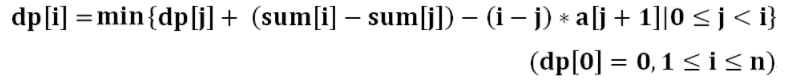因为是排好序的，所以 a [ j + 1 ] a[j+1] a [ j + 1 : i ] a[j+1:i] 这个区间内最小的那个数，这是个 1 D / 1 D 1D/1D 问题，即状态数为 O ( n ) O(n) ，每个状态的转移成本为 O ( n ) O(n) ，所以总的算法复杂度为 O ( n 2 ) O(n^2) 。朴素算法如下：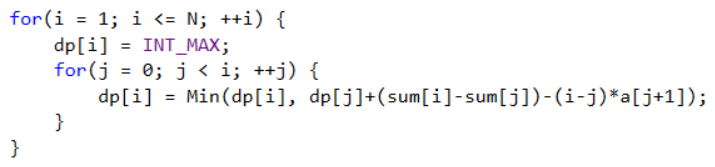由于 n n 的范围太大，需要优化。算法优化的本质就是让上述代码的第一层循环不变，减少第二层循环的状态转移过程。首先将状态转移方程进行移项，得到如下等式：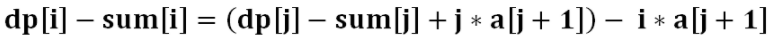看上去貌似并没有什么变化，这么做的目的是什么呢？再来仔细看下这个式子：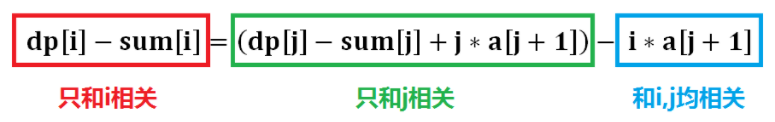这样一看，基本上就能看懂分类依据了，红色代表自变量为i的函数，绿色代表自变量为j的函数，蓝色则是以 i i j j 为变量的函数，那么我们做进一步转化，使得该公式更加清晰。将 i i j j 为自变量的函数分别用b和y表示， k = i k=i 代表斜率。于是，状态转移方程转化成了以 a [ j + 1 ] a[j+1] 为自变量， i i 为斜率， d p [ i ] − s u m [ i ] dp[i]-sum[i] 为截距的直线方程。如上图所示，当 i i 确定， j j t t 时，则代表的是一条斜率为 i i ，并且过点 T ( a [ t + 1 ] , d p [ t ] − s u m [ t ] + t ∗ a [ t + 1 ] ) T(a[t+1], dp[t]-sum[t]+t*a[t+1]) 的直线。更加一般地，当 i i 确定， j j 取不同值时，则这个平面上会出现一系列平行的直线，他们的斜率都是 i i 。因为 i i 是确定的，并且所有直线的斜率就是 i i ，所以所有直线相互平行；又由于 j j 的不同，直线经过不同的点，所以只要是两条不重合的平行直线，截距必然不同，而截距 b = d p [ i ] − s u m [ i ] b=dp[i]-sum[i] ，即 d p [ i ] = b + s u m [ i ] dp[i]=b+sum[i] ；所以可以得出结论：
dp[i]的最小值一定出现在最下面的那条直线上（因为最下面的直线截距最小）。
接下来，我们分析几条比较重要的性质：
第一条：维护“下凸”折线如图所示，A、B、C三个点，其中B点位于AC直线之上，即为一个“上凸”点。然后我们连接AC两点，绘制所有的斜率小于K(A,C)的直线（图中绿色直线），发现截距最小的一定是经过点A的直线（想象一下，图中三根绿色的直线一起动起来的情况）；然后绘制所有的斜率大于K(A,C)的直线（图中红色直线），发现截距最小的一定是经过C的直线。于是，可以得出结论，在用A、B、C进行状态转移时，B永远是一个无用的状态转移。
由于任意三点不能出现“上凸”。所以，所有用于状态转移的点必须是一条“下凸”折线，如图所示：图中每个点都代表了向dp[i]进行状态转移的dp[j]。从图中可以看出，“下凸”折线满足每段折线斜率单调递增。那么如何维护这段“下凸”折线？
我们采用双端队列来维护，由于它存储结构的单调性（斜率单调），又称为“单调队列”。deq[]代表单调队列的数据域，headtail分别表示它的两个指针，当 headtail相等则代表单调队列为空，初始化head=tail=0deq[]中的每个元素存的是原数组的下标，因为通过下标就能计算出坐标图中点的 x-y坐标，也就能计算相邻两点之间的斜率了。
那么每次我们将一个可行的转移状态j放入单调队列之前，先判断它的加入能否保证斜率单调递增，如果不能则将单调队列尾元素删除，依次往复，最后将这个点放入单调队列尾部，这样一条“下凸”折线就算维护完毕了。
细心的读者，会发现这一步操作同样可以用“栈”这种数据结构来完成。接下来，我们继续来探讨为什么用双端队列不用栈。
第二条：“永不录用”点的剔除
“永不录用”这个名字是我自己起的，那么哪些点是“永不录用”点？，还是来看图：由于每次枚举状态i时是递增的枚举（之前那段代码的第一个for循环），也就是说斜率一定是越来越大，那么从图中可以看出，那些斜率小于i的折线上的点（灰色点）如果要经过斜率为i的直线（图中绿色直线的平行线），则产生的截距必然不会比图中绿色直线的更小，也就是灰色点都可以删除了。
这些可以删除的灰色点就是“永不录用”点。“永不录用”点更加官方的说法，就是单调队列里的折线中，所有斜率小于i的折线的左端点。由于单调队列的斜率单调性，所以删除点的操作可以从左往右进行，这就是为什么之前不用栈来维护的根本原因。如果维护折线用的是栈，则最左边的点势必在栈底，而栈这种数据结构只能获取栈顶元素。

# 二、数据结构

️《算法和数据结构》之《画解数据结构》总纲，算法零基础教程️相信看我文章的大多数都是「 大学生 」，能上大学的都是「 精英 」，那么我们自然要「 精益求精 」，如果你还是「 大一 」，那么太好了，你拥有大把时间，当然你可以选择「 刷剧 」，然而，「 学好算法 」，三年后的你自然「 不能同日而语 」
那么这里，我整理了「 几十个基础算法 」 的分类，点击开启：

如果链接被屏蔽，或者有权限问题，可以私聊作者解决。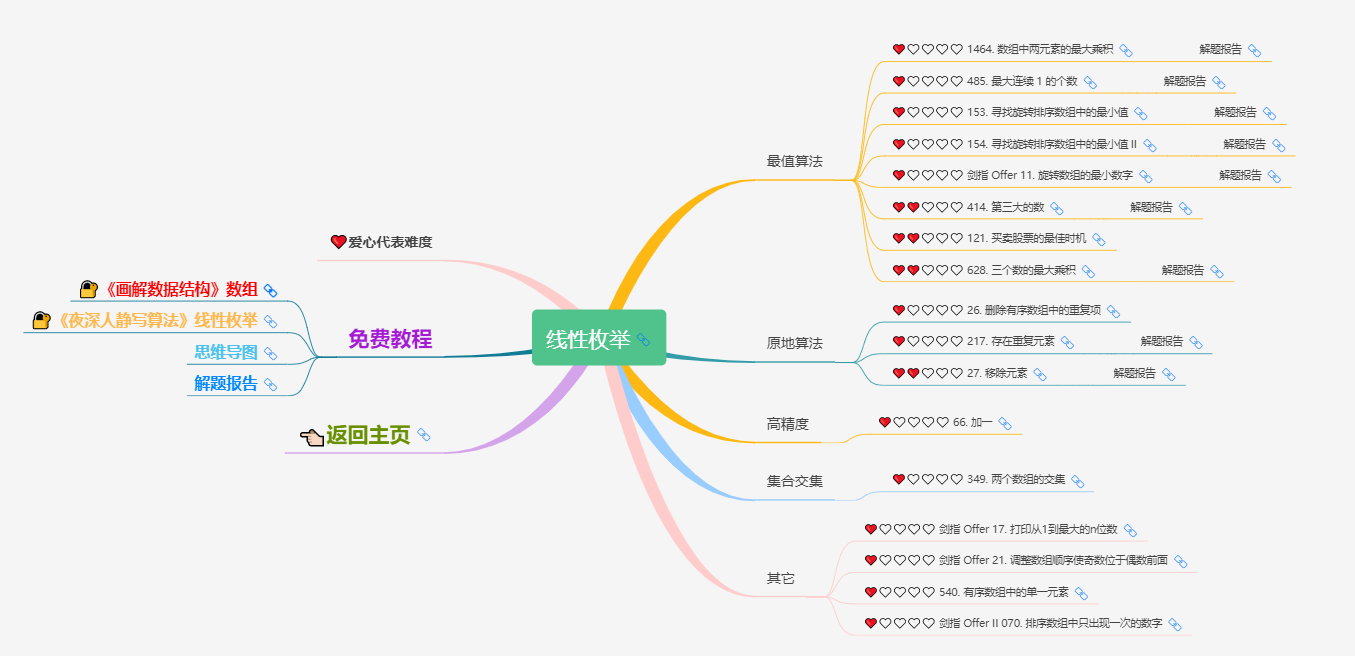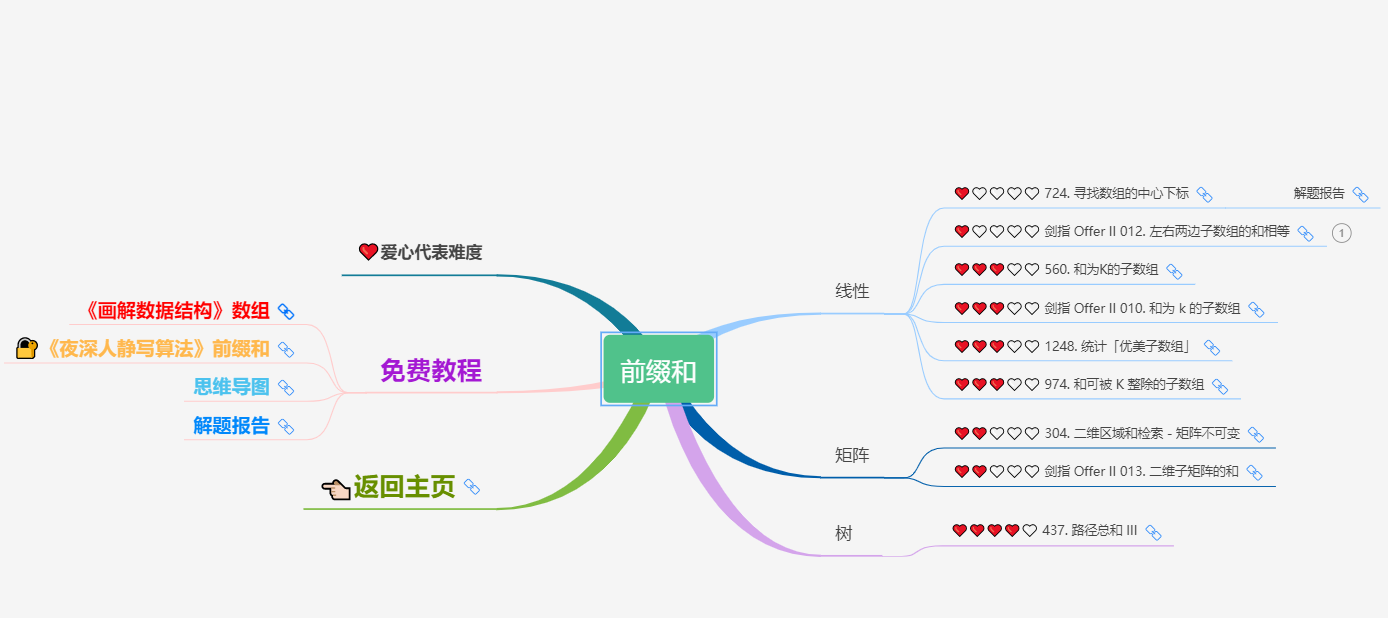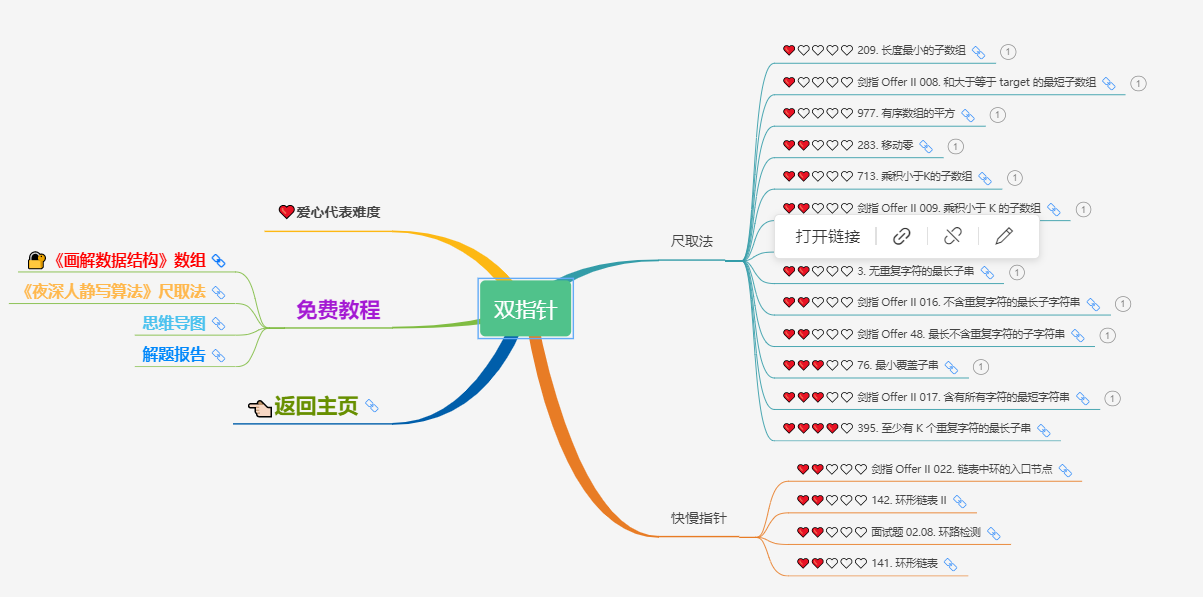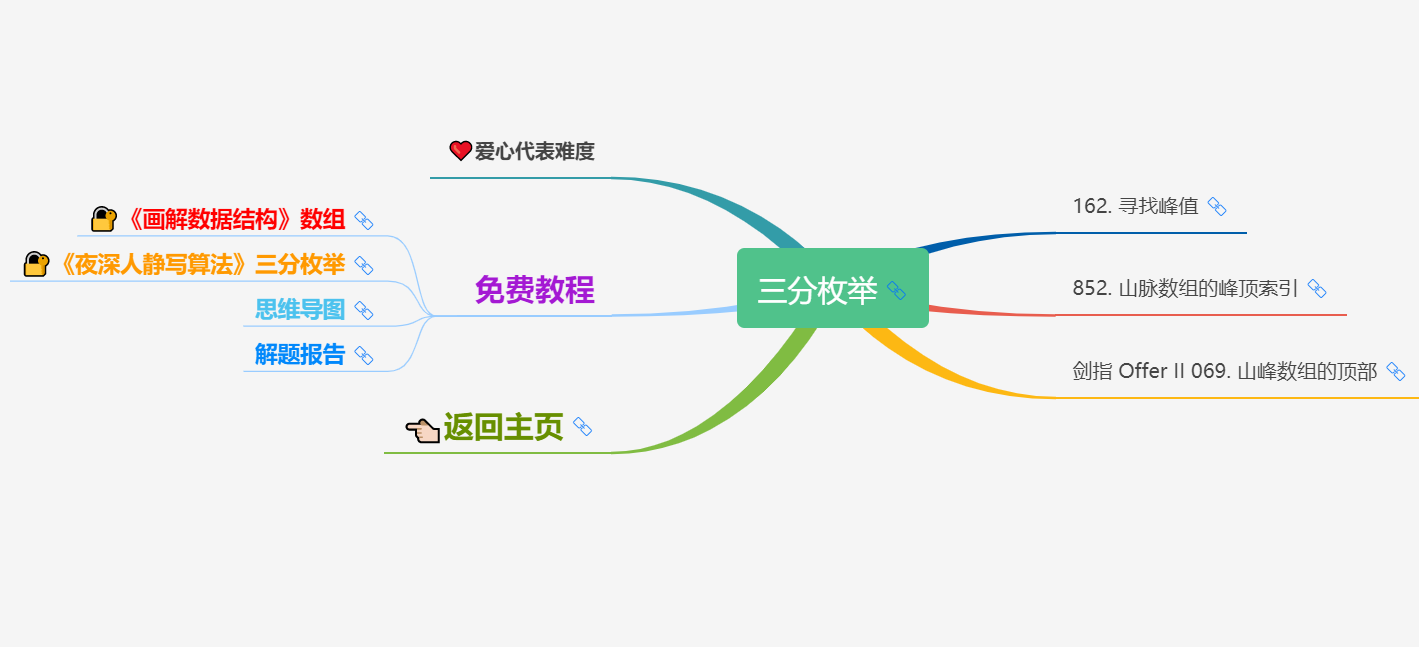C语言免费动漫教程，和我一起打卡！

C语言入门100例

# 粉丝专属福利

### 2019年塑造未来趋势的10个数据预测_10个数据预测方法-程序员宅基地

2019年塑造未来趋势的10个数据预测人们经常会看到一些科技行业炒作周期的兴衰，其中包括网络时代、云计算、大数据，以及最近的人工智能(AI)和区块链的出现。回顾过去，很明显这些重大变化中的每一个都是附加的，或者在某种程度上与之前发生的技术颠覆有关。例如，如果没有大数据，人工智能就不会是今天的样子。如果没有云计算的出现，大数据是不可能实现的，如果没有上世纪90年代互联网的繁荣发展，云计算本身也将不..._10个数据预测方法

### 无锡，满城桂花香_370524316 csdn-程序员宅基地

12日晚上8点，Ｇ215达到无锡东站。刚一出车门，就闻到浓浓的桂花香。每年这个时候，无锡满城都是桂花香，实在是让人陶醉。　　出了站口，一阵莫名的悲伤。曾经，吾在这里接她……..._370524316 csdn

### uniapp input 标签 修改 placeholder 颜色-程序员宅基地

<input placeholder-style="color:rgba(0, 0, 0, 0.4);" placeholder="输入手机号"></input>